### The Glasses Are Off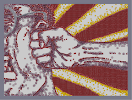Hover over the thumbnail for a full-size version.

Author espada777777 author:espada777777 harryandthepotter nuke palemoon rated 2009-09-23 2009-09-23 4 by 287 people. \$The Glasses Are Off#espada777777#harryandthepotter#00000000000000000000000000000000000000000000000000000000000000000000000000000000000000000000000000000000000000000000000000000000000000000000000000000000000000000000000000000000000000000000000000000000000000000000000000000000000000000000000000000000000000000000000000000000000000000000000000000000000000000000000000000000000000000000000000000000000000000000000000000000000000000000000000000000000000000000000000000000000000000000000000000000000000000000000000000000000000000000000000000000000000000000000000000000000000000000000000000000000000000000000000000000000000000000000000000000000000000000000000000000000000000000000000000000000000000000000000000000000000000000000000000000000000000000000000000000000000000|12^389,412!12^388,412!12^385,413!12^384,412!12^381,411!12^376,411!12^373,411!12^369,412!12^364,410!12^357,411!12^353,411!12^348,412!12^343,413!12^337,411!12^333,411!12^329,411!12^327,411!12^322,410!12^319,410!12^317,410!12^310,408!12^305,408!12^301,407!12^295,406!12^290,406!12^284,405!12^282,405!12^277,405!12^274,405!12^270,406!12^266,406!12^264,405!12^261,404!12^258,402!12^256,401!12^254,398!12^252,396!12^249,394!12^247,392!12^246,392!12^243,390!12^247,384!12^243,386!12^238,387!12^233,387!12^230,386!12^224,382!12^223,381!12^220,379!12^220,377!12^219,373!12^219,369!12^218,365!12^217,363!12^217,360!12^217,357!12^217,355!12^218,353!12^219,351!12^220,349!12^249,383!12^253,382!12^255,381!12^259,381!12^262,381!12^266,381!12^270,380!12^273,379!12^276,378!12^279,377!12^282,377!12^283,377!12^302,373!12^305,370!12^306,367!12^306,363!12^309,359!12^310,358!12^310,356!12^310,355!12^311,352!12^311,350!12^309,346!12^309,344!12^309,342!12^307,338!12^305,336!12^301,336!12^298,332!12^271,393!12^273,391!12^274,388!12^276,385!12^277,382!12^268,395!12^269,398!12^305,382!12^308,391!12^310,398!12^315,371!12^319,386!12^323,393!12^325,398!12^330,405!12^320,354!12^326,366!12^333,373!12^344,388!12^348,395!12^355,399!12^357,402!12^359,407!12^283,385!12^224,345!12^221,346!12^218,346!12^214,347!12^210,347!12^208,347!12^204,345!12^201,343!12^199,342!12^198,340!12^195,337!12^194,335!12^193,334!12^193,333!12^192,328!12^192,325!12^191,320!12^191,316!12^191,314!12^191,311!12^192,308!12^192,307!12^193,307!12^193,303!12^224,343!12^229,343!12^233,343!12^236,341!12^240,340!12^244,340!12^249,338!12^253,338!12^258,337!12^261,336!12^265,335!12^267,333!12^270,332!12^273,331!12^278,329!12^282,328!12^292,326!12^295,324!12^288,325!12^292,331!12^290,331!12^285,332!12^297,323!12^302,321!12^304,321!12^306,319!12^307,316!12^308,314!12^308,313!12^309,311!12^309,307!12^311,303!12^311,301!12^312,298!12^313,294!12^313,291!12^312,287!12^311,285!12^311,283!12^308,282!12^306,281!12^298,279!12^296,279!12^291,280!12^300,280!12^194,298!12^393,413!12^398,413!12^401,411!12^406,410!12^409,409!12^414,407!12^415,406!12^415,403!12^420,405!12^425,403!12^429,401!12^432,399!12^433,397!12^434,394!12^435,393!12^436,391!12^439,388!12^441,386!12^445,382!12^454,378!12^450,372!12^449,371!12^451,378!12^459,377!12^463,376!12^425,405!12^427,404!12^432,403!12^437,403!12^443,402!12^446,401!12^451,401!12^449,399!12^452,398!12^456,395!12^461,391!12^469,388!12^471,387!12^471,385!12^465,392!12^461,397!12^465,397!12^471,397!12^476,396!12^480,393!12^483,392!12^485,391!12^491,389!12^495,388!12^499,386!12^505,385!12^509,384!12^514,384!12^518,384!12^284,376!12^288,375!12^292,375!12^295,372!12^298,373!12^244,283!12^245,284!12^251,287!12^255,288!12^289,282!12^286,285!12^283,285!12^279,284!12^277,284!12^272,284!12^269,283!12^295,280!12^301,279!12^304,277!12^305,274!12^307,270!12^307,268!12^308,267!12^308,265!12^308,261!12^309,257!12^309,254!12^307,249!12^307,249!12^306,246!12^244,281!12^241,280!12^240,276!12^239,275!12^237,272!12^235,266!12^235,262!12^236,260!12^237,258!12^237,254!12^237,253!12^235,251!12^236,247!12^236,246!12^236,243!12^235,240!12^235,237!12^235,234!12^235,232!12^235,231!12^233,227!12^233,223!12^232,222!12^231,218!12^230,214!12^229,210!12^229,207!12^229,204!12^230,201!12^231,199!12^232,197!12^234,195!12^237,194!12^239,194!12^241,192!12^244,190!12^247,189!12^250,187!12^255,185!12^259,185!12^263,184!12^266,184!12^268,184!12^271,182!12^278,181!12^281,180!12^288,179!12^291,179!12^294,178!12^295,178!12^297,177!12^299,177!12^302,177!12^257,286!12^261,284!12^263,284!12^266,281!12^269,278!12^270,277!12^273,274!12^276,273!12^277,271!12^278,268!12^281,262!12^284,260!12^285,258!12^285,256!12^286,253!12^288,250!12^289,246!12^291,243!12^293,241!12^293,240!12^294,237!12^292,234!12^292,230!12^290,228!12^289,226!12^283,219!12^283,219!12^297,236!12^299,236!12^301,235!12^303,235!12^305,237!12^306,238!12^308,239!12^312,238!12^316,235!12^316,232!12^318,230!12^319,228!12^320,225!12^320,223!12^320,222!12^321,237!12^323,238!12^330,235!12^335,233!12^338,231!12^342,223!12^343,221!12^318,241!12^316,243!12^317,247!12^318,250!12^320,253!12^322,257!12^323,259!12^324,261!12^327,264!12^328,267!12^329,270!12^330,271!12^332,275!12^334,276!12^339,277!12^342,278!12^335,281!12^334,282!12^335,285!12^335,288!12^336,291!12^340,298!12^344,303!12^348,308!12^350,310!12^354,313!12^359,316!12^363,320!12^368,322!12^373,322!12^377,322!12^382,323!12^384,323!12^388,326!12^393,327!12^397,328!12^401,328!12^406,328!12^410,326!12^414,323!12^406,333!12^410,334!12^415,335!12^420,336!12^426,336!12^241,240!12^243,240!12^247,240!12^249,240!12^252,241!12^256,243!12^258,245!12^260,247!12^261,251!12^263,251!12^262,254!12^264,256!12^269,265!12^265,256!12^266,262!12^267,264!12^267,266!12^267,268!12^265,270!12^264,274!12^263,277!12^244,254!12^248,253!12^313,243!12^319,239!12^342,234!12^347,233!12^350,234!12^298,174!12^300,172!12^304,172!12^305,172!12^307,171!12^313,171!12^317,171!12^320,172!12^325,170!12^327,169!12^331,169!12^335,169!12^339,168!12^341,166!12^346,165!12^349,164!12^353,163!12^354,162!12^355,161!12^359,162!12^360,162!12^363,162!12^367,163!12^370,163!12^372,163!12^375,164!12^381,169!12^382,170!12^384,171!12^387,175!12^390,178!12^392,180!12^398,183!12^401,186!12^406,190!12^408,193!12^411,197!12^415,199!12^417,201!12^421,204!12^423,206!12^425,209!12^426,210!12^430,212!12^433,214!12^435,217!12^462,235!12^463,234!12^468,236!12^471,239!12^474,241!12^477,243!12^479,245!12^481,247!12^486,252!12^488,252!12^488,252!12^489,252!12^490,253!12^437,219!12^441,220!12^444,221!12^448,224!12^451,225!12^453,227!12^458,231!12^463,233!12^465,235!12^469,237!12^491,253!12^492,253!12^496,254!12^499,254!12^505,253!12^508,254!12^512,254!12^515,253!12^517,253!12^523,252!12^532,251!12^535,251!12^540,251!12^546,250!12^548,250!12^553,250!12^559,251!12^564,252!12^567,253!12^573,253!12^579,253!12^586,253!12^594,254!12^596,255!12^600,254!12^604,254!12^610,254!12^611,254!12^616,255!12^621,255!12^624,254!12^629,254!12^633,254!12^639,254!12^643,254!12^649,253!12^653,252!12^657,252!12^661,251!12^666,251!12^669,251!12^671,249!12^677,249!12^682,249!12^685,250!12^688,250!12^696,249!12^706,248!12^707,248!12^711,247!12^715,247!12^719,247!12^723,247!12^724,247!12^729,246!12^731,246!12^736,245!12^740,244!12^742,244!12^747,243!12^749,243!12^754,243!12^756,243!12^759,242!12^764,242!12^767,242!12^770,242!12^772,241!12^481,398!12^483,398!12^488,397!12^496,396!12^500,396!12^506,397!12^510,397!12^514,397!12^519,397!12^527,398!12^530,398!12^534,397!12^537,397!12^541,397!12^544,397!12^550,397!12^555,396!12^561,396!12^572,397!12^577,398!12^581,399!12^587,398!12^593,398!12^601,399!12^609,398!12^614,398!12^620,400!12^624,402!12^630,402!12^636,404!12^642,406!12^649,407!12^662,408!12^670,410!12^675,411!12^678,411!12^684,411!12^688,411!12^693,412!12^697,414!12^702,414!12^712,417!12^720,418!12^723,418!12^728,420!12^734,421!12^738,421!12^742,422!12^748,423!12^752,424!12^757,424!12^761,424!12^764,424!12^767,425!12^771,425!12^704,416!12^709,415!12^710,416!12^716,417!12^655,405!12^660,405!12^665,406!12^654,408!12^661,410!12^666,410!12^668,411!12^567,395!12^594,397!12^602,396!12^691,248!12^703,248!12^524,250!12^527,251!12^195,293!12^190,292!12^188,291!12^187,289!12^184,286!12^183,285!12^182,282!12^180,279!12^180,276!12^179,275!12^179,271!12^179,270!12^179,267!12^180,262!12^180,259!12^182,258!12^182,255!12^184,251!12^186,248!12^187,244!12^187,241!12^188,239!12^189,237!12^194,237!12^198,240!12^201,239!12^204,239!12^207,239!12^211,239!12^214,240!12^218,239!12^217,238!12^219,238!12^223,237!12^226,237!12^228,235!12^228,235!12^230,235!12^200,294!12^203,295!12^207,295!12^210,294!12^213,294!12^215,292!12^219,293!12^225,291!12^227,291!12^229,290!12^232,290!12^237,290!12^239,289!12^240,289!12^242,287!12^244,287!12^245,286!12^246,289!12^248,291!12^250,291!12^253,289!12^191,236!12^188,233!12^186,231!12^184,230!12^183,228!12^183,226!12^183,220!12^183,218!12^182,216!12^182,212!12^184,208!12^183,204!12^183,204!12^183,201!12^185,201!12^190,198!12^190,197!12^193,194!12^195,191!12^198,189!12^201,185!12^204,182!12^204,180!12^206,179!12^208,178!12^210,178!12^212,177!12^215,176!12^275,166!12^273,167!12^275,166!12^290,164!12^293,165!12^297,165!12^301,163!12^305,163!12^275,166!12^279,167!12^282,168!12^283,169!12^284,169!12^286,167!12^291,165!12^297,162!12^300,161!12^304,160!12^308,160!12^308,159!12^313,157!12^316,156!12^319,156!12^322,157!12^325,157!12^329,159!12^332,161!12^335,160!12^338,164!12^339,164!12^342,164!12^238,188!12^230,186!12^240,186!12^246,186!12^238,181!12^238,180!12^238,181!12^237,182!12^235,182!12^232,181!12^241,181!12^247,183!12^216,174!12^218,174!12^223,173!12^224,172!12^227,171!12^229,170!12^231,170!12^234,170!12^238,172!12^241,171!12^243,170!12^246,170!12^248,170!12^250,170!12^252,169!12^256,169!12^259,169!12^263,168!12^266,169!12^268,171!12^271,175!12^271,178!12^276,170!12^273,169!12^256,174!12^258,176!12^257,178!12^261,178!12^263,179!12^285,174!12^123,174!12^118,179!12^115,184!12^112,190!12^108,195!12^105,201!12^103,209!12^101,215!12^100,220!12^100,223!12^99,232!12^99,239!12^98,250!12^99,256!12^102,262!12^104,269!12^105,278!12^96,269!12^93,280!12^88,299!12^87,308!12^88,313!12^88,317!12^88,325!12^88,330!12^90,335!12^93,342!12^94,350!12^96,356!12^100,364!12^103,374!12^104,380!12^106,383!12^108,388!12^110,395!12^114,403!12^117,407!12^120,411!12^122,417!12^124,418!12^128,419!12^150,205!12^146,217!12^142,238!12^153,213!12^153,209!12^157,202!12^160,196!12^169,186!12^171,180!12^164,180!12^158,173!12^172,161!12^150,351!12^158,401!12^182,429!12^275,460!12^275,462!12^276,466!12^272,491!12^277,472!12^277,461!12^278,444!12^276,461!12^276,466!12^276,472!12^275,479!12^275,482!12^274,489!12^269,497!12^266,501!12^262,507!12^255,514!12^251,519!12^245,526!12^241,530!12^239,533!12^235,538!12^233,542!12^230,545!12^226,548!12^223,551!12^221,554!12^220,557!12^214,561!12^212,563!12^208,566!12^204,569!12^200,572!12^200,572!12^311,433!12^311,433!12^312,437!12^312,440!12^312,446!12^311,451!12^312,457!12^313,461!12^314,467!12^314,475!12^317,481!12^318,487!12^319,491!12^321,497!12^322,503!12^323,508!12^324,514!12^325,518!12^326,521!12^327,527!12^329,532!12^330,537!12^332,543!12^333,547!12^334,551!12^336,555!12^338,559!12^340,562!12^342,564!12^345,568!12^347,574!12^349,575!12^310,430!12^310,426!12^309,425!12^308,422!12^308,418!12^308,416!12^307,414!12^140,431!12^147,436!12^155,438!12^160,439!12^166,444!12^165,444!12^155,440!12^170,453!12^159,450!12^159,453!12^159,462!12^158,471!12^161,463!12^197,453!12^225,449!12^226,450!12^232,448!12^213,450!12^215,452!12^217,481!12^220,485!12^320,331!12^315,324!12^314,316!12^314,312!12^316,309!12^315,308!12^325,277!12^328,287!12^328,295!12^327,300!12^326,296!12^324,285!12^324,278!12^325,274!12^326,268!12^307,268!12^312,260!12^312,256!12^311,251!12^311,249!12^475,365!12^476,362!12^478,359!12^480,358!12^485,354!12^488,353!12^502,353!12^481,355!12^488,356!12^496,352!12^497,351!12^501,349!12^509,349!12^515,348!12^521,347!12^527,347!12^533,347!12^538,347!12^542,347!12^546,346!12^551,346!12^557,345!12^564,344!12^573,344!12^578,343!12^585,344!12^590,346!12^595,347!12^599,348!12^603,348!12^611,348!12^616,348!12^622,349!12^628,350!12^640,352!12^650,354!12^664,358!12^684,365!12^693,365!12^714,366!12^723,368!12^732,369!12^741,370!12^750,371!12^753,371!12^504,316!12^509,317!12^514,318!12^529,319!12^532,319!12^537,319!12^546,320!12^551,320!12^559,320!12^568,320!12^574,320!12^582,321!12^595,322!12^603,322!12^612,320!12^616,320!12^495,288!12^499,290!12^505,293!12^511,294!12^519,295!12^534,295!12^541,295!12^559,294!12^474,330!12^474,325!12^475,319!12^446,321!12^448,320!12^452,320!12^453,319!12^438,289!12^446,286!12^457,280!12^464,275!12^451,282!12^420,294!12^415,294!12^506,374!12^511,372!12^515,371!12^528,369!12^536,368!12^545,368!12^556,367!12^569,367!12^575,367!12^582,369!12^590,369!12^595,371!12^602,371!12^608,371!12^619,372!12^630,372!12^636,373!12^648,375!12^655,375!12^633,397!12^225,380!12^185,211!12^183,219!12^236,221!12^239,221!12^242,222!12^247,226!12^238,226!12^307,245!12^299,166!12^307,166!12^314,166!12^364,223!12^372,218!12^376,214!12^360,235!12^366,234!12^374,233!12^375,233!12^340,248!12^388,238!12^399,235!12^376,245!12^374,335!12^376,339!12^380,347!12^388,355!12^393,358!12^396,360!12^402,362!12^424,364!12^425,361!12^441,355!12^384,391!12^395,399!12^399,402!12^405,404!12^417,405!12^523,370!12^560,365!12^567,365!12^573,366!12^605,370!12^612,370!12^617,372!12^626,372!12^632,372!12^638,373!12^632,380!12^636,375!12^642,377!12^646,379!12^652,379!12^691,320!12^720,322!12^759,323!12^681,320!12^664,320!12^137,512!12^128,532!12^116,543!12^126,536!12^135,515!12^137,508!12^130,525!12^43,475!12^43,475!12^50,475!12^61,475!12^68,477!12^73,478!12^75,478!12^79,477!12^79,478!12^93,475!12^96,473!12^98,470!12^98,469!12^84,476!12^70,477!12^54,474!12^46,473!12^42,472!12^39,472!12^30,471!12^28,471!12^95,462!12^99,453!12^97,446!12^96,442!12^95,439!12^91,436!12^88,433!12^87,433!12^73,431!12^65,430!12^57,430!12^48,429!12^45,429!12^40,431!12^36,432!12^33,432!12^27,431!12^30,432!12^66,429!12^72,430!12^82,429!12^87,432!12^65,529!12^55,545!12^53,555!12^53,557!12^58,546!12^65,539!12^72,532!12^66,531!12^55,549!12^53,559!12^50,563!12^48,563!12^46,558!12^40,555!12^72,565!12^82,568!12^68,426!12^68,423!12^68,421!12^68,420!12^67,414!12^66,408!12^66,406!12^68,401!12^66,401!12^64,400!12^62,400!12^58,401!12^56,401!12^50,400!12^49,399!12^48,399!12^45,400!12^44,402!12^42,404!12^42,406!12^42,408!12^40,411!12^40,414!12^40,419!12^39,423!12^39,425!12^39,426!12^39,403!12^37,402!12^34,399!12^32,397!12^30,397!12^29,397!12^30,398!12^47,387!12^46,387!12^49,386!12^49,386!12^50,389!12^50,393!12^49,395!12^47,395!12^49,386!12^49,381!12^49,378!12^50,372!12^50,371!12^50,368!12^49,368!12^47,367!12^47,365!12^47,364!12^47,364!12^62,347!12^61,352!12^58,356!12^54,358!12^50,360!12^44,364!12^36,362!12^34,362!12^28,363!12^24,363!12^58,345!12^58,343!12^57,336!12^54,336!12^49,335!12^45,335!12^40,335!12^34,333!12^30,332!12^26,332!12^56,363!12^57,361!12^59,365!12^59,367!12^60,373!12^61,381!12^63,385!12^66,386!12^67,388!12^70,394!12^73,397!12^75,399!12^76,404!12^78,407!12^79,412!12^81,416!12^82,421!12^82,426!10^76,424!10^76,422!10^72,414!10^72,410!10^72,408!10^72,408!10^73,423!10^64,392!10^61,393!10^58,394!10^56,393!10^56,389!10^56,384!10^55,377!10^54,374!10^53,368!10^51,392!10^55,379!10^55,372!10^54,366!12^68,344!12^65,339!12^62,336!12^56,331!12^54,326!12^53,321!12^54,313!12^54,309!12^55,305!12^55,301!12^53,300!12^52,311!12^50,322!12^50,328!12^61,335!12^68,343!12^72,347!12^74,349!12^76,351!12^36,269!12^40,250!12^39,243!12^40,233!12^46,223!12^50,217!12^45,226!12^40,239!12^42,245!12^41,253!12^45,256!12^45,263!12^45,267!12^36,290!12^34,293!12^31,296!12^27,298!12^38,287!12^41,276!12^42,267!12^44,273!12^43,287!12^43,287!12^37,293!12^35,142!12^39,142!12^41,140!12^45,138!12^48,137!12^53,134!12^56,133!12^58,133!12^62,132!12^65,128!12^66,128!12^67,127!12^70,124!12^70,124!12^39,140!12^36,140!12^32,139!12^25,139!12^25,138!12^65,122!12^62,121!12^52,120!12^48,120!12^45,121!12^39,123!12^36,123!12^34,122!12^29,122!12^24,121!12^24,121!12^57,121!12^57,121!12^68,120!12^66,118!12^64,117!12^61,116!12^50,109!12^44,109!12^43,109!12^42,109!12^39,108!12^35,107!12^30,106!12^29,106!12^27,104!12^26,104!12^25,104!12^67,125!12^70,126!12^73,129!12^74,130!12^78,133!12^83,136!12^86,135!12^89,134!12^82,115!12^83,113!12^88,112!12^91,110!12^98,110!12^72,108!12^73,102!12^78,98!12^82,96!12^85,95!12^88,93!12^68,142!12^68,143!12^70,148!12^72,150!12^73,151!12^55,150!12^50,149!12^54,147!12^50,150!12^44,154!12^39,156!12^29,157!12^23,156!12^34,154!12^45,187!12^43,183!12^48,174!12^54,169!12^58,168!12^57,167!12^47,181!12^46,184!12^111,156!12^109,142!12^108,136!12^108,133!12^109,129!12^112,123!12^115,115!12^118,108!12^118,108!12^113,116!12^111,124!12^259,513!12^259,512!12^55,111!12^57,112!12^61,115!12^28,88!12^29,88!12^38,87!12^41,87!12^46,87!12^50,86!12^53,86!12^56,87!12^58,87!12^62,87!12^66,85!12^70,85!12^74,84!12^79,83!12^82,83!12^85,81!12^91,80!12^95,78!12^101,72!12^106,68!12^107,65!12^108,62!12^111,55!12^114,48!12^111,76!12^114,74!12^119,71!12^124,65!12^127,63!12^124,86!12^123,88!12^127,88!12^34,87!12^35,60!12^28,75!12^28,80!12^31,66!12^34,62!12^39,59!12^56,53!12^46,64!12^44,72!12^40,77!12^45,62!12^51,56!12^48,67!12^55,58!12^55,59!12^56,68!12^60,59!12^58,69!12^61,73!12^75,53!12^74,54!12^56,86!12^63,62!12^72,66!12^76,68!12^80,64!12^87,48!12^86,51!12^72,77!12^42,71!12^37,72!12^33,70!12^36,61!12^33,58!12^28,56!12^30,55!12^49,53!12^58,51!12^71,52!12^93,49!12^92,62!12^91,62!12^96,56!12^100,54!12^111,48!12^104,57!12^97,63!12^97,55!12^113,43!12^118,44!12^122,46!12^125,44!12^97,44!12^93,46!12^95,149!12^97,137!12^102,119!12^104,109!12^83,54!12^75,48!12^100,75!2^25,210,-0.707106781186547,-0.707106781186547!2^28,209,-0.707106781186547,-0.707106781186547!2^32,209,-0.707106781186547,-0.707106781186547!2^36,208,-0.707106781186547,-0.707106781186547!2^40,207,-0.707106781186547,-0.707106781186547!2^42,206,-0.707106781186547,-0.707106781186547!2^44,205,-0.707106781186547,-0.707106781186547!2^46,203,-0.707106781186547,-0.707106781186547!2^50,201,-0.707106781186547,-0.707106781186547!2^52,199,-0.707106781186547,-0.707106781186547!2^55,199,-0.707106781186547,-0.707106781186547!2^57,197,-0.707106781186547,-0.707106781186547!2^61,195,-0.707106781186547,-0.707106781186547!2^62,193,-0.707106781186547,-0.707106781186547!2^65,192,-0.707106781186547,-0.707106781186547!2^67,191,-0.707106781186547,-0.707106781186547!2^71,187,-0.707106781186547,-0.707106781186547!2^73,185,-0.707106781186547,-0.707106781186547!2^80,177,-0.707106781186547,-0.707106781186547!2^84,172,-0.707106781186547,-0.707106781186547!2^88,167,-0.707106781186547,-0.707106781186547!2^88,164,-0.707106781186547,-0.707106781186547!2^88,162,-0.707106781186547,-0.707106781186547!2^90,158,-0.707106781186547,-0.707106781186547!2^91,156,-0.707106781186547,-0.707106781186547!2^91,154,-0.707106781186547,-0.707106781186547!2^93,150,-0.707106781186547,-0.707106781186547!2^93,147,-0.707106781186547,-0.707106781186547!2^94,145,-0.707106781186547,-0.707106781186547!2^95,141,-0.707106781186547,-0.707106781186547!2^95,139,-0.707106781186547,-0.707106781186547!2^94,137,-0.707106781186547,-0.707106781186547!2^94,133,-0.707106781186547,-0.707106781186547!2^95,128,-0.707106781186547,-0.707106781186547!2^95,123,-0.707106781186547,-0.707106781186547!2^95,119,-0.707106781186547,-0.707106781186547!2^94,117,-0.707106781186547,-0.707106781186547!2^94,114,-0.707106781186547,-0.707106781186547!2^94,110,-0.707106781186547,-0.707106781186547!2^93,107,-0.707106781186547,-0.707106781186547!2^94,104,-0.707106781186547,-0.707106781186547!2^93,101,-0.707106781186547,-0.707106781186547!2^92,98,-0.707106781186547,-0.707106781186547!2^91,96,-0.707106781186547,-0.707106781186547!2^90,94,-0.707106781186547,-0.707106781186547!2^90,91,-0.707106781186547,-0.707106781186547!2^89,88,-0.707106781186547,-0.707106781186547!2^88,84,-0.707106781186547,-0.707106781186547!2^88,83,-0.707106781186547,-0.707106781186547!2^87,81,-0.707106781186547,-0.707106781186547!2^85,79,-0.707106781186547,-0.707106781186547!2^82,77,-0.707106781186547,-0.707106781186547!2^82,74,-0.707106781186547,-0.707106781186547!2^80,72,-0.707106781186547,-0.707106781186547!2^79,69,-0.707106781186547,-0.707106781186547!2^76,66,-0.707106781186547,-0.707106781186547!2^74,65,-0.707106781186547,-0.707106781186547!2^72,63,-0.707106781186547,-0.707106781186547!2^71,61,-0.707106781186547,-0.707106781186547!2^68,57,-0.707106781186547,-0.707106781186547!2^67,56,-0.707106781186547,-0.707106781186547!2^65,55,-0.707106781186547,-0.707106781186547!2^61,53,-0.707106781186547,-0.707106781186547!2^59,52,-0.707106781186547,-0.707106781186547!2^57,49,-0.707106781186547,-0.707106781186547!2^49,48,-0.707106781186547,-0.707106781186547!2^44,47,-0.707106781186547,-0.707106781186547!2^43,47,-0.707106781186547,-0.707106781186547!2^39,47,-0.707106781186547,-0.707106781186547!2^37,47,-0.707106781186547,-0.707106781186547!2^35,47,-0.707106781186547,-0.707106781186547!2^31,46,-0.707106781186547,-0.707106781186547!2^29,46,-0.707106781186547,-0.707106781186547!2^26,47,-0.707106781186547,-0.707106781186547!2^26,47,-0.707106781186547,-0.707106781186547!2^23,48,-0.707106781186547,-0.707106781186547!12^204,172!12^204,168!12^204,165!12^204,161!12^207,157!12^209,157!12^211,153!12^212,150!12^217,147!12^220,144!12^223,141!12^225,138!12^227,135!12^230,131!12^235,127!12^237,125!12^238,122!12^239,119!12^242,116!12^245,116!12^248,115!12^253,116!12^255,118!12^260,120!12^264,122!12^268,126!12^271,128!12^276,131!12^279,133!12^282,134!12^290,136!12^294,145!12^294,149!12^294,152!12^294,156!12^293,159!12^294,154!12^295,147!12^293,142!12^231,160!12^231,160!12^234,156!12^237,152!12^239,148!12^242,144!12^242,142!12^245,141!12^248,141!12^252,140!12^254,140!12^261,140!12^265,142!12^266,143!12^269,146!12^271,147!12^275,150!12^276,153!12^276,158!12^275,159!12^253,157!12^256,153!12^258,152!12^261,148!12^259,153!12^253,155!12^250,156!12^244,159!12^318,150!12^319,147!12^321,145!12^322,141!12^322,135!12^321,131!12^319,126!12^320,119!12^318,112!12^317,108!12^317,102!12^316,97!12^316,94!12^315,90!12^316,84!12^316,80!12^315,75!12^314,71!12^314,65!12^313,58!12^312,55!12^312,51!12^310,50!12^308,46!12^305,42!12^303,38!12^300,33!12^297,26!12^295,23!12^293,22!2^102,105,0,-1!2^105,103,0,-1!2^107,102,0,-1!2^112,101,0,-1!2^117,100,0,-1!2^121,99,0,-1!2^124,98,0,-1!2^128,98,0,-1!2^131,97,0,-1!2^134,96,0,-1!2^136,96,0,-1!2^139,95,0,-1!2^141,94,0,-1!2^143,93,0,-1!2^146,92,0,-1!2^149,91,0,-1!2^152,89,0,-1!2^156,88,0,-1!2^158,87,0,-1!2^162,86,0,-1!2^165,85,0,-1!2^170,84,0,-1!2^172,85,0,-1!2^175,83,0,-1!2^179,83,0,-1!2^183,82,0,-1!2^188,81,0,-1!2^189,81,0,-1!2^192,79,0,-1!2^196,78,0,-1!2^201,77,0,-1!2^206,76,0,-1!2^211,75,0,-1!2^213,75,0,-1!2^217,75,0,-1!2^226,73,0,-1!2^228,73,0,-1!2^231,74,0,-1!2^235,74,0,-1!2^240,74,0,-1!2^243,74,0,-1!2^246,75,0,-1!2^250,76,0,-1!2^255,77,0,-1!2^259,78,0,-1!2^263,80,0,-1!2^266,83,0,-1!2^268,85,0,-1!2^271,91,0,-1!2^272,96,0,-1!2^273,98,0,-1!2^273,103,0,-1!2^273,107,0,-1!2^272,109,0,-1!2^271,111,0,-1!2^271,113,0,-1!2^270,116,0,-1!12^313,150!12^314,138!12^317,129!12^317,140!12^317,143!12^312,132!12^312,125!12^311,138!12^310,141!12^306,125!12^303,124!12^320,132!12^324,140!12^327,147!12^327,149!12^319,137!12^323,124!12^326,132!12^330,137!12^324,110!12^322,109!12^331,124!12^328,121!12^322,107!12^321,104!12^326,106!12^319,90!12^324,93!12^326,108!12^329,112!12^322,91!12^322,85!12^318,78!12^319,75!12^325,83!12^324,84!12^322,75!12^320,64!12^319,50!12^317,33!12^315,30!12^316,38!12^313,41!12^306,24!12^311,34!12^306,27!12^308,33!12^310,40!12^311,53!12^313,66!12^311,83!12^312,107!12^314,118!12^308,109!12^308,97!12^305,116!12^303,92!12^305,87!12^301,111!12^305,89!12^301,107!12^294,98!12^298,117!12^300,126!12^299,139!12^307,146!12^305,142!12^303,152!12^303,147!12^305,134!12^302,131!12^302,136!12^301,132!12^294,129!12^290,128!12^281,124!12^279,124!12^271,120!12^283,120!12^282,118!12^282,117!12^283,107!12^287,100!12^287,94!12^287,88!12^288,112!12^291,121!12^292,116!12^294,98!12^282,90!12^283,83!12^279,76!12^276,73!12^273,70!12^269,68!12^267,65!12^261,64!12^252,64!12^249,64!12^246,62!12^256,65!12^261,67!12^264,67!12^258,113!12^259,109!12^257,103!12^259,96!12^259,93!12^256,83!12^253,82!12^246,81!12^240,80!12^235,80!12^254,87!12^250,97!12^254,102!12^252,104!12^246,91!12^246,84!12^242,97!12^242,103!12^240,108!12^237,116!12^245,109!12^247,109!12^235,111!12^233,119!12^226,127!12^226,131!12^229,122!12^231,112!12^234,102!12^239,94!12^236,95!12^228,110!12^228,120!12^226,127!12^222,130!12^217,136!12^212,141!12^205,145!12^204,149!12^192,158!12^192,161!12^207,152!12^197,165!12^196,175!12^192,182!12^192,165!12^199,153!12^207,131!12^215,118!12^218,112!12^228,102!12^219,112!12^218,125!12^212,130!12^213,127!12^225,102!12^226,95!12^230,93!12^236,87!12^231,85!12^230,78!12^230,78!12^221,83!12^214,92!12^212,110!12^209,115!12^210,121!12^220,102!12^218,92!12^217,90!12^209,90!12^209,87!12^208,83!12^215,82!12^217,80!12^222,80!12^221,94!12^220,107!12^217,110!12^211,96!12^292,103!12^296,101!12^302,99!12^303,99!12^298,77!12^289,88!12^297,93!12^297,92!12^295,83!12^291,80!12^289,75!12^284,69!12^289,72!12^299,80!12^303,80!12^310,71!12^307,61!12^306,58!12^305,78!12^302,62!12^302,49!12^299,45!12^304,67!12^304,68!12^302,70!12^293,63!12^294,62!12^282,61!12^276,61!12^279,65!12^281,57!12^302,55!12^301,46!12^297,55!12^294,57!12^289,51!12^293,46!12^294,39!12^291,25!12^291,43!12^282,57!12^276,59!12^282,45!12^286,34!12^285,38!12^270,55!12^268,56!12^275,40!12^280,34!12^280,30!12^269,49!12^265,52!12^257,51!12^253,50!12^266,30!12^271,17!12^260,33!12^254,40!12^256,44!12^270,35!12^278,27!12^273,38!12^265,48!12^258,54!12^253,64!12^253,61!12^268,60!12^280,66!12^286,51!12^277,46!12^271,45!12^264,42!12^246,62!12^241,64!12^236,62!12^232,62!12^227,63!12^223,64!12^218,65!12^213,66!12^204,64!12^202,65!12^197,64!12^196,62!12^189,57!12^188,55!12^187,51!12^183,44!12^177,39!12^175,38!12^169,33!12^163,29!12^159,28!12^152,25!12^146,23!12^166,23!12^181,29!12^187,37!12^191,43!12^193,48!12^178,36!12^175,33!12^191,42!12^197,52!12^204,55!12^216,55!12^207,51!12^196,43!12^201,40!12^216,51!12^229,54!12^237,54!12^247,55!12^226,54!12^221,56!12^209,54!12^200,54!12^192,34!12^187,25!12^211,42!12^213,57!12^211,58!12^214,61!12^219,59!12^231,53!12^248,51!12^246,42!12^227,41!12^218,41!12^208,27!12^208,29!12^222,45!12^231,42!12^243,45!12^218,35!12^205,31!12^199,35!12^213,35!12^223,33!12^233,39!12^239,40!12^233,30!12^229,29!12^247,29!12^250,33!12^262,28!12^249,29!12^252,27!12^272,32!12^283,30!12^291,55!12^306,57!12^276,18!12^250,22!12^244,25!12^231,25!12^221,22!12^211,28!12^195,24!12^203,29!12^229,27!12^238,25!12^241,25!12^249,37!12^239,33!12^241,40!12^238,45!12^222,40!12^202,38!12^226,45!12^263,38!12^268,32!12^261,28!12^263,26!12^284,29!12^289,30!12^302,34!12^309,31!12^198,30!12^182,29!12^145,24!12^123,21!12^93,26!12^91,24!12^81,25!12^73,25!12^63,23!12^54,20!12^80,22!12^85,25!12^89,27!12^94,24!12^98,23!12^106,21!12^124,19!12^122,24!12^123,24!12^134,25!12^139,23!12^148,23!12^116,23!12^109,21!3^310,272!3^312,275!3^314,275!3^315,265!3^315,260!3^313,259!3^311,256!3^311,253!3^309,251!3^314,264!3^316,266!3^321,272!3^325,275!3^320,277!3^315,270!3^316,277!3^317,280!3^319,280!3^318,287!3^316,294!3^318,300!3^321,292!3^324,288!3^325,282!3^324,287!3^327,297!3^331,301!3^333,299!3^339,302!3^341,304!3^344,307!3^345,308!3^348,312!3^352,316!3^355,318!3^358,321!3^367,323!3^374,324!3^378,326!3^386,330!3^392,330!3^397,332!3^401,333!3^404,335!3^370,329!3^369,332!3^369,335!3^372,341!3^374,345!3^378,348!3^382,351!3^385,355!3^391,359!3^396,362!3^362,330!3^360,327!3^356,321!3^353,316!3^298,327!3^301,329!3^304,327!3^308,326!3^311,322!3^316,310!3^316,308!3^312,323!3^313,334!3^313,337!3^315,348!3^312,352!3^316,344!3^319,334!3^319,328!3^324,318!3^319,329!3^322,335!3^326,336!3^330,338!3^334,341!3^316,359!3^314,360!3^313,364!3^312,367!3^310,372!3^312,372!3^315,362!3^311,337!3^309,335!3^304,332!3^309,329!3^315,320!3^315,310!3^316,302!3^316,300!3^321,331!3^322,313!3^323,305!3^321,346!3^320,356!3^322,366!3^326,375!3^335,385!3^339,389!3^344,392!3^350,399!3^354,402!3^311,377!3^317,381!3^316,388!3^317,393!3^319,395!3^321,399!3^302,375!3^301,385!3^301,389!3^299,393!3^295,374!3^300,381!3^294,382!3^291,382!3^285,384!3^279,387!3^277,394!3^254,388!3^256,388!3^266,386!3^269,386!3^257,386!3^255,390!3^258,392!3^261,396!3^273,398!3^284,398!3^302,403!3^308,403!3^330,407!3^336,408!3^342,407!3^345,407!3^367,403!3^366,404!3^380,403!3^386,405!3^391,406!3^399,406!3^386,399!3^382,396!3^380,392!3^378,387!3^374,383!3^373,380!3^404,403!3^417,402!3^440,397!3^442,399!3^445,391!3^447,388!3^442,398!3^446,392!3^450,387!3^456,383!3^461,380!3^466,379!3^480,397!3^474,394!3^475,392!3^482,384!3^492,397!3^492,395!3^496,394!3^504,394!3^507,393!3^513,391!3^516,390!3^521,390!3^523,389!3^525,393!3^534,392!3^538,392!3^544,392!3^549,393!3^555,391!3^559,389!3^565,390!3^573,388!3^580,389!3^594,389!3^600,389!3^607,387!3^611,387!3^618,390!3^622,391!3^634,392!3^639,391!3^642,392!3^649,393!3^655,393!3^665,393!3^670,392!3^677,391!3^688,393!3^700,396!3^708,398!3^713,399!3^718,400!3^727,401!3^732,402!3^737,402!3^746,401!3^749,402!3^752,403!3^757,404!3^761,405!3^769,406!3^620,396!3^620,395!3^631,395!3^643,396!3^645,397!3^658,397!3^662,399!3^666,401!3^676,401!3^679,403!3^686,403!3^696,403!3^703,404!3^717,407!3^726,408!3^742,412!3^750,413!3^757,414!3^767,414!3^767,415!3^753,419!3^741,416!3^735,414!3^731,413!3^719,408!3^711,407!3^692,404!3^684,402!3^675,399!3^672,399!3^700,398!3^705,403!3^710,405!3^721,406!3^735,408!3^746,409!3^754,410!3^767,408!3^771,415!3^763,418!3^750,416!3^737,413!3^728,413!3^751,406!3^764,402!3^778,395!3^761,399!3^750,398!3^738,396!3^717,392!3^701,390!3^688,390!3^672,390!3^651,385!3^650,385!3^661,384!3^670,386!3^692,392!3^708,390!3^631,398!3^606,393!3^594,391!3^576,390!3^629,386!3^624,380!3^605,377!3^650,401!3^646,399!3^659,400!3^669,402!3^678,405!3^691,408!3^697,408!3^703,406!3^708,407!3^733,411!3^749,410!3^508,374!3^520,375!3^526,374!3^531,373!3^537,372!3^544,371!3^550,371!3^563,370!3^580,370!3^545,378!3^533,381!3^530,387!3^568,375!3^579,377!3^593,379!3^597,379!3^616,380!3^640,380!3^644,382!3^632,381!3^620,381!3^475,364!3^476,362!3^485,370!3^488,368!3^487,365!3^493,362!3^498,360!3^486,365!3^485,368!3^493,362!3^497,360!3^505,359!3^514,357!3^517,357!3^528,356!3^535,356!3^543,355!3^555,354!3^561,354!3^564,352!3^574,354!3^586,353!3^593,354!3^614,356!3^620,358!3^630,361!3^641,363!3^655,364!3^684,366!3^697,369!3^710,371!3^727,372!3^741,374!3^744,374!3^748,374!3^755,376!3^760,382!3^759,381!3^768,389!3^758,388!3^745,386!3^764,377!3^771,376!3^508,310!3^509,310!3^514,311!3^518,312!3^528,315!3^541,317!3^545,317!3^553,318!3^558,317!3^569,317!3^578,318!3^588,317!3^597,315!3^604,315!3^660,317!3^672,317!3^686,318!3^579,326!3^584,326!3^596,324!3^606,323!3^614,323!3^571,324!3^560,323!3^558,321!3^716,320!3^740,323!3^743,323!3^766,321!3^500,282!3^506,284!3^508,287!3^516,289!3^526,288!3^534,289!3^541,290!3^545,289!3^549,289!3^550,289!3^569,288!3^579,290!3^586,290!3^554,257!3^560,258!3^549,254!3^544,254!3^534,254!3^510,254!3^503,256!3^559,255!3^568,257!3^575,257!3^582,258!3^589,259!3^596,259!3^602,259!3^608,260!3^622,261!3^629,261!3^635,259!3^645,259!3^658,260!3^664,260!3^671,259!3^683,258!3^690,257!3^695,256!3^710,255!3^724,255!3^730,254!3^737,253!3^743,253!3^754,251!3^768,249!3^770,249!3^606,264!3^621,262!3^625,262!3^640,260!3^648,260!3^658,261!3^664,261!3^671,262!3^680,263!3^689,262!3^696,261!3^710,261!3^718,263!3^728,263!3^741,261!3^752,260!3^763,258!3^767,258!3^750,263!3^729,257!3^710,258!3^741,255!3^756,250!3^717,273!3^745,275!3^767,272!3^733,276!3^693,273!3^664,271!3^632,270!3^618,268!3^673,345!3^705,288!3^653,275!3^647,281!3^622,282!3^599,276!3^542,266!3^513,262!3^493,266!3^482,260!3^484,267!3^470,271!3^468,273!3^464,280!3^452,287!3^458,283!3^463,282!3^470,279!3^473,276!3^476,274!3^482,270!3^495,263!3^498,261!3^492,260!3^502,261!3^468,266!3^476,255!3^469,241!3^471,251!3^469,248!3^463,241!3^449,236!3^414,328!3^421,322!3^423,321!3^341,283!3^343,287!3^346,284!3^351,283!3^362,284!3^325,248!3^327,250!3^325,244!3^330,240!3^337,240!3^344,241!3^355,240!3^358,240!3^371,237!3^328,243!3^328,249!3^332,257!3^333,263!3^333,268!3^337,273!3^320,231!3^324,226!3^325,223!3^343,235!3^349,227!3^350,223!3^364,229!3^373,224!3^292,220!3^294,221!3^297,227!3^302,232!3^304,234!3^306,234!3^297,251!3^299,243!3^299,243!3^303,250!3^304,264!3^300,247!3^299,246!3^301,252!3^303,262!3^301,273!3^293,265!3^285,275!3^280,276!3^284,273!3^290,263!3^292,255!3^292,254!3^298,285!3^304,289!3^308,296!3^304,309!3^303,314!3^297,319!3^292,322!3^284,324!3^281,326!3^275,326!3^263,327!3^259,332!3^255,334!3^246,336!3^239,337!3^229,339!3^224,341!3^281,337!3^302,339!3^305,347!3^304,357!3^301,362!3^296,367!3^292,369!3^287,371!3^276,372!3^268,372!3^260,374!3^253,375!3^248,377!3^246,379!3^244,381!3^241,381!3^226,373!3^226,376!3^232,379!3^236,381!3^226,364!3^223,358!3^223,354!3^244,383!3^253,388!3^233,365!3^210,337!3^205,334!3^211,334!3^203,332!3^197,324!3^196,319!3^198,312!3^199,305!3^204,302!3^209,299!3^209,331!3^213,334!3^212,293!3^220,290!3^226,289!3^230,289!3^236,289!3^243,290!3^209,288!3^204,288!3^197,289!3^187,284!3^182,282!3^192,243!3^195,242!3^199,245!3^205,246!3^212,244!3^217,243!3^225,244!3^227,244!3^228,247!3^249,234!3^242,231!3^239,229!3^250,233!3^256,238!3^259,240!3^234,217!3^234,210!3^237,210!3^239,207!3^242,213!3^248,220!3^252,224!3^242,202!3^246,199!3^252,197!3^255,195!3^243,248!3^242,260!3^243,266!3^243,269!3^244,272!3^245,250!3^256,251!3^273,266!3^278,260!3^284,250!3^283,237!3^268,248!3^224,324!3^194,228!3^193,224!3^191,208!3^194,202!3^197,197!3^202,193!3^207,188!3^211,183!3^215,182!3^221,181!3^208,188!3^211,188!3^221,189!3^228,186!3^235,187!3^203,188!3^210,188!3^223,187!3^230,187!3^225,180!3^234,177!3^247,177!3^252,180!3^248,177!3^266,174!3^271,174!3^280,173!3^285,173!3^291,173!3^297,171!3^297,171!3^319,165!3^329,168!3^330,168!3^397,186!3^402,189!3^407,197!3^408,203!3^411,208!3^419,210!3^427,213!3^385,178!3^314,177!3^324,176!3^330,177!3^334,178!3^340,176!3^346,168!3^261,402!3^153,457!3^150,467!3^152,471!3^155,459!3^156,452!3^152,450!3^145,444!3^140,443!3^128,439!3^121,433!3^137,436!3^127,428!3^120,421!3^114,415!3^105,405!3^104,391!3^99,380!3^95,370!3^93,359!3^91,346!3^87,339!3^86,334!3^83,325!3^80,316!3^79,301!3^114,409!3^125,434!3^131,436!3^142,441!3^148,448!3^147,459!3^139,450!3^137,450!3^153,471!3^148,485!3^144,492!3^187,459!3^207,462!3^217,460!3^223,457!3^202,464!3^199,463!3^226,451!3^216,473!3^221,482!3^143,516!3^140,521!3^126,527!3^127,518!3^131,508!3^124,519!3^122,526!3^115,530!3^56,553!3^50,543!3^43,548!3^35,552!3^46,544!3^58,535!3^55,533!3^49,544!3^48,554!3^42,556!3^49,560!3^49,567!3^36,568!3^34,570!3^41,573!3^54,571!3^57,570!3^73,570!3^77,575!3^51,570!3^38,576!3^36,577!3^32,567!3^29,562!3^31,550!3^32,496!3^30,499!3^32,492!3^46,486!3^60,486!3^68,485!3^48,483!3^38,483!3^36,483!3^30,479!3^28,482!3^35,489!3^40,486!3^53,482!3^66,482!3^68,483!3^35,486!3^49,487!3^61,483!3^75,483!3^86,484!3^92,482!3^99,474!3^103,450!3^93,503!3^81,512!3^61,504!3^207,571!3^207,571!3^211,567!3^223,564!3^229,555!3^236,546!3^247,531!3^253,524!3^256,520!3^261,514!3^272,505!3^278,495!3^278,470!3^276,470!3^278,478!3^278,489!3^277,494!3^275,507!3^271,516!3^265,524!3^259,531!3^255,537!3^254,544!3^250,549!3^246,559!3^241,569!3^239,571!3^221,571!3^217,582!3^228,576!3^233,569!3^235,559!3^240,546!3^245,538!3^246,534!3^235,550!3^229,558!3^220,564!3^216,569!3^212,572!3^224,563!3^237,552!3^248,542!3^244,541!3^230,565!3^229,575!3^250,545!3^252,554!3^243,576!3^253,567!3^259,542!3^260,545!3^263,573!3^257,569!3^271,537!3^276,569!3^287,577!3^276,570!3^353,573!3^342,571!3^333,568!3^309,575!3^296,572!3^327,570!3^330,556!3^324,535!3^321,528!3^324,556!3^318,561!3^314,577!3^320,544!3^319,525!3^318,505!3^314,486!3^310,474!3^309,467!3^306,471!3^308,427!3^308,421!3^305,418!3^307,453!3^305,437!3^300,423!3^297,419!3^287,414!3^267,412!3^275,416!3^291,417!3^297,422!3^285,424!3^296,425!3^291,428!3^274,426!3^268,426!3^268,423!3^289,423!3^275,413!3^284,415!3^293,412!3^299,412!3^277,412!3^264,413!3^260,417!3^248,410!3^246,405!3^244,404!3^241,401!3^240,400!3^236,398!3^238,398!3^242,396!3^259,417!3^250,412!3^265,422!3^271,429!3^288,433!3^290,446!3^304,455!3^303,465!3^287,436!3^269,430!3^256,428!3^239,409!3^231,405!3^227,397!3^257,434!3^264,441!3^243,435!3^238,417!3^228,404!3^234,391!3^208,353!3^183,346!3^189,357!3^198,349!3^182,342!3^176,325!3^177,296!3^176,285!3^172,273!3^176,254!3^179,241!3^182,232!3^180,228!3^177,224!3^173,211!3^172,203!3^175,196!3^180,191!3^187,185!3^196,178!3^180,194!3^179,195!3^187,179!3^193,172!3^195,171!3^194,161!3^194,159!3^196,157!3^193,177!3^195,171!3^198,160!3^203,155!3^202,392!3^188,378!3^206,407!3^212,418!3^179,438!3^188,441!3^216,440!3^197,445!3^192,444!3^205,447!3^209,448!3^93,278!3^94,270!3^95,258!3^88,250!3^88,237!3^93,245!3^91,234!3^90,219!3^92,209!3^103,193!3^110,181!3^88,264!3^83,264!3^75,281!3^60,279!3^43,325!3^48,314!3^48,306!3^46,314!3^45,323!3^42,327!3^30,329!3^26,333!3^27,329!3^39,318!3^39,316!3^34,314!3^26,315!3^24,307!3^25,302!3^30,315!3^35,323!3^33,323!3^39,324!3^42,323!3^29,303!3^34,299!3^41,294!3^46,288!3^47,282!3^49,272!3^50,258!3^48,261!3^49,252!3^49,242!3^50,236!3^34,281!3^29,289!3^25,296!3^29,287!3^28,273!3^27,265!3^51,349!3^49,411!3^48,415!3^46,420!3^45,425!3^51,426!3^58,426!3^33,426!3^29,425!3^27,422!3^28,406!3^28,370!3^27,372!3^32,370!3^34,370!3^40,370!3^28,375!3^26,384!3^61,325!3^62,326!3^67,354!3^66,359!3^67,369!3^70,380!3^71,385!3^68,381!3^63,366!3^64,363!3^67,359!3^75,393!3^83,405!3^87,412!3^90,423!3^93,437!3^99,449!3^82,387!3^86,379!3^90,375!3^91,401!3^96,427!3^103,441!3^198,554!3^184,564!3^177,568!3^170,569!3^177,570!3^184,570!3^194,571!3^198,564!3^204,561!3^214,555!3^211,554!3^171,571!3^165,573!3^155,571!3^133,571!3^127,571!3^118,571!3^108,572!3^103,573!3^95,573!3^94,571!3^105,562!3^115,549!3^116,557!3^138,568!3^150,572!3^103,571!3^85,574!3^72,575!3^130,559!3^174,467!3^217,496!3^216,500!3^207,512!3^203,515!3^267,458!3^266,453!3^275,454!3^28,231!3^30,223!3^42,217!3^44,213!3^27,218!3^32,218!3^45,217!3^47,216!3^51,210!3^55,208!3^56,206!3^60,204!3^63,204!3^66,201!3^68,197!3^72,194!3^92,167!3^96,158!3^101,150!3^103,144!3^78,191!3^84,183!3^86,179!3^88,175!3^92,172!3^58,211!3^64,209!3^60,219!3^65,213!3^81,197!3^87,192!3^83,205!3^88,213!3^75,207!3^83,213!3^98,187!3^101,175!3^103,136!3^103,128!3^105,122!3^106,118!3^106,118!3^107,119!3^104,145!3^103,158!3^106,165!3^107,171!3^91,180!3^82,191!3^78,189!3^82,196!3^32,161!3^27,165!3^26,166!3^28,174!3^26,177!3^38,161!3^45,160!3^51,158!3^37,177!3^37,183!3^41,186!3^40,172!3^47,164!3^29,189!3^26,194!3^64,150!3^68,152!3^70,153!3^24,134!3^28,134!3^34,135!3^36,136!3^39,136!3^45,132!3^53,130!3^59,127!3^25,109!3^29,110!3^36,113!3^50,116!3^50,117!3^30,150!3^29,153!3^31,155!3^41,150!3^26,168!3^28,176!3^29,182!3^30,172!3^36,167!3^42,164!3^24,95!3^30,92!3^28,100!3^33,100!3^39,96!3^42,95!3^52,93!3^60,93!3^67,91!3^70,90!3^68,92!3^70,93!3^69,96!3^65,103!3^66,105!3^34,98!3^41,97!3^44,97!3^49,94!3^54,92!3^33,104!3^45,102!3^54,105!3^62,114!3^74,128!3^26,75!3^27,63!3^27,69!3^28,77!3^39,78!3^44,76!3^51,77!3^36,57!3^44,63!3^50,75!3^55,79!3^64,83!3^44,189!3^58,181!3^66,176!3^78,152!3^41,191!3^37,192!3^75,131!3^74,121!3^74,112!3^80,131!3^82,106!3^73,154!3^61,169!3^61,73!3^66,75!3^55,70!3^50,66!3^28,27!3^24,32!3^28,30!3^40,27!3^47,28!3^40,35!3^54,34!3^57,36!3^63,39!3^70,43!3^56,26!3^64,32!3^72,37!3^87,59!3^90,60!3^97,67!3^100,66!3^103,51!3^103,48!3^101,86!3^106,76!3^110,69!3^112,62!3^107,79!3^106,89!3^107,90!3^115,86!3^119,84!3^110,110!3^109,111!3^117,108!3^123,104!3^128,103!3^135,101!3^145,101!3^149,100!3^152,100!3^154,101!3^156,99!3^161,98!3^166,98!3^171,98!3^177,98!3^181,98!3^187,97!3^191,97!3^196,97!3^200,97!3^206,97!3^209,97!3^213,98!3^220,97!3^226,98!3^229,99!3^235,101!3^239,106!3^240,106!3^243,111!3^122,103!3^134,102!3^140,102!3^153,101!3^163,100!3^184,98!3^194,98!3^120,119!3^122,116!3^130,112!3^139,110!3^151,108!3^161,106!3^171,105!3^192,102!3^207,100!3^220,99!3^194,108!3^196,122!3^200,134!3^206,109!3^211,101!3^232,98!3^240,112!3^229,109!3^228,104!3^227,104!3^232,106!3^236,109!3^238,113!3^238,114!3^175,102!3^183,104!3^197,115!3^200,108!3^201,125!3^197,150!3^191,160!3^184,166!3^188,111!3^188,118!3^191,129!3^192,146!3^211,120!3^215,119!3^215,133!3^210,143!3^207,144!3^218,137!3^223,129!3^223,120!3^220,113!3^221,115!3^223,119!3^225,122!3^225,124!3^152,98!3^159,97!3^163,97!3^167,98!3^173,97!3^184,97!3^191,97!3^199,97!3^205,99!3^210,99!3^216,99!3^23,41!3^25,54!3^23,55!3^19,47!3^19,42!3^39,27!3^40,25!3^76,24!3^86,40!3^80,38!3^78,28!3^91,27!3^105,28!3^111,26!3^118,26!3^124,25!3^133,25!3^141,26!3^163,93!3^176,90!3^188,90!3^196,91!3^204,90!3^200,83!3^191,64!3^189,58!3^174,69!3^176,65!3^178,53!3^169,39!3^158,35!3^141,29!3^137,28!3^125,24!3^130,27!3^140,28!3^148,32!3^165,33!3^154,24!3^163,25!3^166,27!3^177,30!3^184,36!3^189,42!3^191,48!3^191,47!3^184,26!3^184,24!3^194,24!3^204,24!3^212,23!3^212,28!3^213,25!3^209,18!3^202,13!3^206,12!3^237,17!3^203,51!3^201,53!3^204,45!3^207,42!3^214,40!3^224,35!3^230,34!3^216,61!3^208,63!3^216,58!3^232,56!3^247,60!3^246,61!3^231,54!3^232,37!3^245,30!3^256,33!3^263,38!3^272,42!3^278,47!3^286,51!3^291,54!3^295,59!3^114,165!3^117,167!3^122,168!3^128,163!3^133,158!3^145,156!3^154,153!3^161,151!3^170,150!3^140,124!3^115,151!3^119,153!3^129,152!3^128,150!3^116,136!3^117,146!3^102,160!3^100,155!3^98,141!3^100,134!3^103,131!3^102,169!3^95,178!3^91,183!3^108,118!3^109,118!3^110,117!3^108,114!3^106,127!3^105,138!3^102,144!3^101,150!3^99,156!3^96,165!3^94,171!3^89,179!3^84,190!3^112,179!3^172,155!3^182,160!3^178,150!3^187,136!3^173,138!3^165,143!3^169,119!3^184,131!3^179,134!3^170,126!3^167,193!3^95,281!3^99,289!3^104,294!3^109,305!3^82,296!3^159,432!3^181,412!3^172,421!3^170,416!3^169,410!3^172,403!3^171,423!3^176,436!3^156,397!3^163,387!3^162,405!3^156,402!3^168,429!3^222,451!3^240,452!3^231,457!3^241,462!3^242,462!3^242,463!3^243,469!3^225,479!3^168,530!3^156,511!3^165,480!3^162,460!3^169,466!3^180,481!3^203,470!3^277,535!3^274,558!3^268,551!3^280,538!3^287,516!3^296,559!3^303,560!3^142,425!3^156,427!3^145,415!3^106,314!3^92,322!3^96,333!3^235,84!3^245,89!3^250,96!3^251,101!3^250,106!3^222,83!3^222,83!3^285,122!3^286,117!3^288,84!3^286,66!3^286,64!3^294,91!3^297,104!3^300,117!3^302,121!3^305,109!3^308,87!3^309,84!3^311,106!3^313,118!3^314,130!3^311,143!3^307,146!3^302,151!3^300,151!3^299,132!3^299,98!3^296,74!3^295,66!3^297,67!3^308,77!3^307,76!3^297,61!3^293,57!3^273,38!3^263,40!3^264,52!3^248,54!3^232,48!3^225,48!3^239,48!3^260,40!3^263,24!3^269,26!3^282,27!3^291,29!3^296,33!3^302,27!3^276,23!3^264,25!3^252,32!3^258,29!3^272,21!3^285,25!3^296,24!3^275,25!3^273,22!3^288,27!3^297,32!3^261,58!3^271,56!3^279,54!3^279,48!3^302,118!3^291,116!3^291,108!3^290,101!3^292,97!3^256,101!3^236,161!3^236,159!3^241,157!3^242,150!3^246,148!3^247,150!3^248,150!3^252,149!3^253,149!3^254,147!3^256,146!3^268,163!3^261,165!3^246,162!3^243,162!3^210,162!3^228,166!3^249,162!3^267,159!3^267,154!3^210,169!3^211,166!3^220,153!3^284,145!3^282,142!3^246,126!3^253,127!3^285,160!0^395,173!0^397,172!0^399,171!0^402,168!0^405,165!0^410,163!0^413,161!0^416,159!0^422,156!0^424,155!0^426,153!0^430,149!0^432,147!0^436,143!0^442,140!0^447,138!0^451,135!0^455,131!0^460,127!0^465,124!0^470,121!0^473,118!0^477,114!0^481,110!0^486,106!0^489,104!0^492,101!0^495,98!0^500,94!0^503,92!0^506,90!0^510,86!0^515,82!0^517,80!0^520,77!0^524,74!0^528,72!0^533,69!0^536,65!0^541,61!0^544,59!0^549,55!0^551,49!0^555,46!0^559,43!0^565,37!0^571,33!0^575,30!0^580,26!0^582,24!0^586,19!0^589,16!0^440,211!0^443,209!0^446,209!0^448,207!0^452,205!0^456,203!0^458,201!0^464,197!0^467,195!0^474,191!0^476,190!0^480,188!0^484,186!0^487,184!0^493,181!0^499,179!0^503,175!0^509,172!0^512,171!0^517,168!0^520,166!0^523,164!0^527,161!0^531,159!0^534,157!0^539,155!0^543,152!0^547,149!0^551,149!0^555,145!0^560,141!0^564,139!0^570,136!0^576,134!0^582,131!0^585,127!0^590,126!0^597,121!0^601,120!0^605,117!0^610,114!0^614,113!0^616,111!0^621,108!0^625,105!0^627,103!0^634,99!0^638,98!0^646,95!0^652,93!0^658,91!0^662,87!0^668,86!0^671,84!0^676,82!0^681,80!0^684,79!0^687,76!0^691,74!0^697,72!0^705,68!0^714,66!0^717,64!0^723,60!0^730,58!0^736,55!0^741,53!0^747,51!0^753,50!0^759,48!0^764,47!0^766,47!0^771,46!0^533,245!0^536,244!0^541,242!0^548,240!0^551,239!0^557,237!0^562,236!0^565,236!0^571,235!0^576,233!0^581,232!0^589,229!0^593,228!0^603,226!0^608,226!0^618,221!0^624,218!0^630,216!0^637,216!0^642,214!0^653,212!0^656,212!0^661,210!0^668,208!0^676,205!0^681,204!0^684,202!0^691,200!0^694,198!0^701,196!0^707,194!0^711,194!0^715,192!0^720,191!0^725,190!0^732,188!0^738,187!0^742,186!0^748,185!0^756,182!0^760,179!0^765,175!0^772,169!0^774,167!0^767,220!0^767,220!0^763,220!0^757,222!0^754,222!0^747,225!0^742,226!0^739,227!0^734,229!0^729,229!0^722,228!0^711,228!0^673,237!0^661,240!0^658,243!0^648,246!0^644,247!0^643,247!0^716,232!0^718,232!0^726,229!0^713,233!0^709,234!0^707,235!0^701,236!0^697,237!0^694,238!0^689,239!0^685,239!0^680,240!0^677,242!0^673,243!0^671,243!0^668,244!0^665,245!0^662,246!0^727,225!0^738,223!0^755,215!0^758,215!0^761,213!0^773,210!0^685,234!0^700,232!0^768,215!0^633,248!0^553,246!0^565,244!0^570,243!0^585,238!0^596,236!0^600,235!0^614,232!0^618,227!0^626,226!0^632,223!0^646,217!0^657,217!0^672,214!0^686,212!0^699,204!0^702,203!0^718,197!0^724,196!0^734,193!0^748,192!0^757,188!0^763,185!0^742,204!0^719,208!0^688,215!0^660,230!0^636,234!0^605,244!0^766,206!0^746,215!0^713,220!0^679,229!0^725,188!0^729,186!0^739,185!0^742,182!0^746,177!0^751,176!0^759,173!0^764,170!0^736,182!0^742,180!0^751,178!0^756,176!0^761,171!0^768,189!0^336,128!0^339,126!0^342,123!0^345,121!0^349,118!0^352,113!0^354,111!0^358,105!0^362,101!0^364,98!0^369,92!0^372,88!0^376,85!0^379,81!0^382,78!0^386,75!0^394,68!0^397,64!0^402,60!0^407,55!0^410,49!0^415,44!0^423,40!0^430,35!0^434,32!0^438,30!0^440,26!0^443,22!0^448,19!0^452,16!0^327,71!0^327,70!0^326,70!0^328,67!0^332,64!0^335,60!0^339,54!0^340,51!0^343,48!0^346,43!0^349,39!0^351,36!0^353,32!0^355,28!0^358,24!0^360,21!0^362,18!0^391,72!0^414,50!0^420,47!0^428,40!0^432,35!0^336,132!0^334,134!0^332,136!0^337,117!0^342,114!0^347,109!0^356,104!0^357,101!0^361,93!0^365,88!0^378,73!0^383,67!0^393,62!0^397,51!0^403,47!0^409,43!0^416,37!0^424,33!0^429,29!0^326,78!0^332,73!0^338,69!0^341,62!0^343,54!0^349,49!0^356,42!0^357,32!0^360,29!0^361,25!0^329,93!0^332,85!0^338,79!0^352,58!0^360,48!0^364,40!0^373,27!0^334,111!0^348,102!0^355,91!0^362,84!0^370,79!0^374,72!0^385,62!0^387,53!0^394,47!0^401,41!0^407,37!0^414,28!0^338,97!0^368,68!0^379,50!0^383,39!0^392,25!0^353,75!0^433,26!0^435,207!0^448,199!0^453,198!0^461,191!0^475,184!0^480,180!0^490,175!0^500,170!0^506,166!0^528,154!0^534,149!0^543,141!0^552,136!0^562,131!0^573,128!0^583,124!0^595,114!0^606,109!0^617,104!0^551,142!0^625,98!0^645,89!0^652,87!0^673,82!0^671,77!0^679,71!0^695,66!0^716,58!0^722,52!0^732,49!0^749,44!0^752,41!0^763,39!0^774,36!0^738,48!0^750,37!0^760,30!0^766,25!0^760,45!0^764,42!0^400,178!0^406,168!0^414,162!0^434,154!0^442,150!0^449,142!0^459,135!0^468,129!0^481,118!0^490,113!0^497,106!0^508,97!0^516,87!0^535,75!0^548,67!0^558,60!0^564,48!0^571,36!0^579,31!0^586,26!0^553,59!0^559,51!0^410,180!0^414,178!0^423,169!0^428,165!0^446,154!0^465,139!0^475,131!0^485,123!0^499,114!0^511,104!0^519,93!0^537,85!0^549,76!0^557,69!0^568,62!0^574,51!0^585,39!0^596,32!0^603,23!0^609,15!0^430,204!0^442,195!0^453,191!0^460,188!0^473,184!0^485,177!0^497,165!0^507,156!0^515,154!0^523,150!0^544,136!0^553,131!0^581,115!0^590,110!0^605,102!0^615,98!0^628,89!0^644,82!0^666,70!0^667,70!0^692,54!0^719,47!0^728,41!0^739,35!0^749,30!0^754,26!0^705,61!0^717,54!0^731,25!0^729,29!0^696,46!0^660,59!0^642,70!0^629,81!0^606,91!0^600,96!0^587,101!0^549,123!0^530,136!0^508,149!0^479,161!0^456,180!0^430,191!0^418,186!0^439,170!0^456,157!0^487,127!0^507,109!0^547,88!0^569,69!0^602,46!0^615,34!0^626,25!0^666,19!0^724,25!0^485,147!0^546,113!0^572,94!0^640,55!0^673,40!0^695,25!0^320,455!0^323,455!0^327,456!0^331,457!0^338,458!0^344,460!0^353,462!0^362,467!0^366,468!0^373,469!0^378,473!0^386,475!0^392,476!0^400,479!0^411,481!0^420,484!0^425,488!0^433,491!0^441,494!0^448,496!0^455,499!0^465,502!0^471,504!0^480,508!0^485,512!0^495,515!0^504,520!0^512,524!0^520,526!0^531,530!0^539,534!0^546,539!0^557,545!0^564,549!0^571,553!0^576,557!0^585,561!0^592,565!0^600,567!0^610,572!0^616,576!0^621,579!0^401,419!0^403,421!0^407,422!0^414,425!0^419,426!0^425,428!0^433,431!0^437,433!0^444,436!0^452,440!0^458,444!0^465,445!0^470,449!0^475,450!0^483,452!0^490,455!0^495,458!0^502,459!0^508,462!0^517,466!0^525,467!0^531,473!0^542,474!0^549,477!0^557,480!0^565,483!0^571,486!0^581,490!0^592,494!0^598,498!0^606,501!0^615,504!0^621,509!0^630,513!0^637,517!0^649,520!0^656,523!0^666,528!0^673,532!0^685,536!0^698,542!0^702,543!0^707,545!0^716,548!0^727,554!0^739,557!0^757,564!0^764,565!0^768,567!0^671,532!0^677,534!0^683,535!0^692,538!0^698,540!0^708,543!0^717,546!0^730,550!0^739,553!0^748,555!0^755,557!0^765,561!0^772,563!0^642,519!0^657,522!0^661,524!0^587,490!0^576,487!0^535,472!0^514,462!0^405,481!0^414,482!0^459,501!0^474,504!0^489,512!0^499,517!0^524,525!0^552,544!0^582,560!0^594,562!0^601,567!0^606,575!0^610,579!0^596,571!0^601,573!0^603,578!0^319,447!0^324,448!0^330,451!0^336,452!0^353,452!0^360,459!0^371,463!0^380,465!0^391,472!0^407,475!0^423,483!0^441,489!0^453,493!0^461,497!0^470,501!0^482,506!0^500,514!0^517,518!0^533,525!0^546,527!0^554,535!0^576,548!0^590,558!0^612,563!0^620,571!0^629,575!0^385,418!0^395,422!0^404,425!0^413,428!0^428,436!0^438,441!0^449,446!0^464,451!0^471,458!0^486,459!0^492,465!0^501,469!0^512,472!0^523,477!0^539,483!0^549,485!0^553,487!0^561,488!0^573,492!0^589,500!0^605,507!0^614,512!0^629,517!0^645,525!0^658,531!0^670,537!0^682,541!0^698,546!0^713,552!0^723,554!0^733,562!0^752,567!0^760,571!0^765,575!0^376,418!0^360,421!0^380,426!0^392,430!0^404,437!0^421,445!0^440,454!0^453,457!0^470,466!0^486,470!0^512,484!0^525,488!0^541,494!0^573,498!0^588,508!0^620,529!0^643,537!0^661,544!0^678,552!0^693,560!0^722,569!0^751,581!0^770,592!0^316,431!0^321,435!0^336,442!0^354,451!0^366,458!0^380,464!0^407,472!0^418,476!0^443,488!0^462,488!0^477,497!0^493,503!0^517,510!0^539,519!0^566,530!0^589,540!0^601,552!0^621,557!0^639,568!0^667,575!0^331,421!0^349,429!0^373,446!0^407,452!0^418,463!0^463,475!0^478,480!0^522,494!0^561,508!0^588,521!0^626,543!0^678,566!0^693,575!0^332,518!0^335,521!0^339,527!0^345,532!0^354,537!0^357,537!0^364,542!0^369,547!0^376,549!0^380,554!0^386,560!0^391,566!0^401,571!0^405,573!0^412,576!0^416,577!0^334,531!0^337,532!0^340,535!0^346,540!0^358,543!0^364,549!0^372,555!0^381,560!0^387,566!0^401,573!0^410,576!0^389,561!0^399,567!0^416,567!0^427,572!0^396,561!0^398,561!0^408,565!0^416,570!0^423,571!0^436,575!0^344,548!0^347,551!0^353,553!0^373,561!0^381,565!0^389,570!0^351,562!0^367,572!0^376,578!0^366,562!0^381,572!0^385,553!0^387,554!0^392,556!0^399,561!0^405,565!0^411,567!0^423,571!0^428,574!0^351,533!0^347,532!0^341,525!0^337,521!12^328,150!12^333,147!12^336,142!12^338,139!12^346,128!12^350,123!12^356,119!12^358,114!12^364,108!12^342,134!12^360,118!12^367,106!12^369,104!12^371,101!12^373,96!12^378,92!12^382,87!12^388,81!12^390,80!12^393,77!12^398,74!12^399,72!12^404,66!12^408,63!12^410,61!12^414,57!12^418,52!12^420,51!12^422,48!12^426,45!12^430,42!12^435,36!12^437,33!12^447,27!12^450,25!12^453,23!12^457,20!12^364,112!12^327,153!12^333,154!12^334,151!12^337,150!12^341,146!12^341,144!12^344,140!12^347,137!12^350,133!12^351,129!12^353,128!12^355,125!12^358,121!12^360,118!12^365,115!12^348,132!12^369,110!12^373,106!12^375,100!12^380,95!12^383,92!12^389,86!12^391,82!12^394,79!12^396,74!12^398,70!12^402,67!12^408,64!12^412,60!12^415,56!12^419,53!12^422,48!12^431,43!12^433,42!12^437,37!12^442,33!12^445,30!12^449,27!12^454,24!12^458,21!12^320,67!12^323,63!12^327,59!12^330,56!12^334,54!12^336,51!12^338,47!12^342,42!12^343,37!12^345,35!12^348,29!12^349,27!12^350,25!12^317,59!12^320,57!12^324,56!12^326,53!12^332,48!12^334,44!12^336,40!12^338,36!12^341,30!12^344,26!12^345,23!12^344,27!12^347,20!12^343,30!12^339,36!12^334,47!12^329,51!12^389,169!12^391,168!12^395,166!12^399,162!12^402,160!12^405,158!12^408,156!12^410,152!12^417,148!12^422,146!12^426,145!12^432,142!12^437,138!12^438,136!12^445,131!12^449,129!12^451,125!12^457,122!12^459,120!12^462,117!12^467,113!12^468,111!12^474,107!12^479,105!12^483,102!12^488,98!12^491,95!12^492,93!12^494,92!12^498,89!12^502,85!12^505,83!12^508,79!12^512,75!12^515,74!12^519,70!12^521,68!12^524,66!12^528,64!12^532,61!12^536,56!12^539,54!12^543,51!12^547,48!12^550,46!12^559,39!12^563,36!12^567,32!12^569,29!12^571,26!12^573,23!12^574,22!12^416,150!12^411,151!12^416,150!12^453,126!12^467,116!12^471,113!12^413,153!12^416,150!12^421,147!12^427,143!12^431,139!12^435,135!12^438,133!12^442,127!12^464,110!12^469,106!12^473,103!12^479,100!12^484,96!12^489,90!12^493,86!12^495,83!12^499,80!12^504,76!12^509,71!12^514,69!12^522,65!12^525,62!12^529,59!12^533,55!12^536,52!12^541,47!12^543,46!12^543,46!12^547,43!12^554,37!12^555,35!12^557,32!12^561,27!12^565,24!12^567,20!12^554,39!12^450,120!12^456,115!12^453,119!12^446,126!12^441,128!12^504,78!12^510,72!12^550,40!12^552,42!12^560,30!12^562,27!12^566,23!12^503,83!12^516,66!12^561,31!12^566,28!12^567,28!12^445,219!12^451,215!12^451,216!12^453,213!12^455,210!12^458,207!12^462,204!12^465,202!12^472,200!12^476,199!12^480,195!12^485,192!12^488,189!12^492,187!12^497,187!12^500,184!12^507,180!12^510,179!12^515,174!12^518,172!12^519,171!12^525,169!12^529,168!12^531,166!12^536,164!12^540,162!12^544,158!12^548,156!12^550,154!12^554,152!12^561,149!12^564,148!12^568,146!12^570,145!12^574,142!12^578,140!12^585,136!12^589,134!12^592,132!12^597,129!12^601,127!12^604,125!12^608,122!12^614,119!12^617,118!12^621,117!12^624,113!12^627,111!12^632,109!12^634,108!12^636,106!12^641,103!12^642,103!12^646,102!12^649,101!12^652,99!12^656,98!12^660,96!12^663,94!12^667,93!12^672,90!12^675,88!12^681,87!12^685,85!12^692,83!12^694,80!12^699,77!12^703,75!12^707,74!12^711,72!12^715,69!12^724,67!12^728,65!12^734,62!12^739,61!12^743,60!12^747,57!12^750,56!12^753,56!12^757,55!12^759,53!12^762,53!12^765,53!12^768,52!12^769,52!12^448,220!12^457,214!12^459,212!12^465,208!12^471,206!12^476,201!12^481,198!12^488,195!12^494,191!12^498,187!12^504,183!12^452,222!12^453,220!12^454,217!12^511,179!12^514,178!12^523,172!12^528,170!12^537,165!12^543,161!12^548,159!12^554,157!12^558,155!12^564,151!12^567,150!12^572,145!12^578,142!12^582,139!12^589,137!12^592,133!12^598,131!12^603,129!12^610,124!12^616,120!12^622,115!12^628,111!12^633,108!12^642,102!12^647,102!12^654,98!12^662,95!12^670,91!12^675,89!12^682,87!12^685,85!12^692,83!12^699,81!12^708,77!12^714,72!12^721,69!12^725,67!12^732,65!12^741,62!12^749,58!12^754,57!12^761,54!12^765,54!12^769,54!12^683,247!12^686,245!12^694,243!12^698,242!12^703,240!12^707,237!12^717,236!12^719,236!12^724,234!12^730,232!12^736,230!12^741,228!12^746,225!12^751,225!12^759,223!12^762,222!12^766,222!12^768,222!12^704,242!12^707,240!12^710,239!12^717,239!12^725,237!12^703,244!12^709,242!12^717,241!12^727,238!12^734,233!12^740,231!12^746,229!12^752,227!12^755,226!12^763,225!12^766,225!12^698,245!12^512,248!12^519,246!12^525,244!12^527,241!12^532,241!12^535,241!12^540,239!12^546,237!12^551,235!12^555,233!12^558,232!12^564,230!12^567,230!12^572,229!12^578,226!12^585,225!12^588,225!12^590,224!12^596,222!12^605,221!12^607,220!12^611,220!12^617,218!12^621,216!12^627,213!12^631,212!12^637,211!12^642,209!12^647,209!12^651,205!12^657,204!12^662,203!12^667,201!12^673,198!12^677,196!12^682,194!12^691,192!12^696,192!12^700,191!12^706,189!12^709,187!12^717,185!12^720,184!12^726,182!12^732,180!12^738,177!12^746,173!12^755,170!12^759,168!12^764,165!12^769,158!12^749,169!12^752,167!12^760,164!12^767,158!12^746,169!12^742,174!12^737,176!12^732,178!12^729,180!12^722,181!12^713,184!12^704,188!12^693,191!12^684,192!12^680,194!12^673,197!12^665,200!12^657,202!12^646,204!12^642,206!12^636,208!12^630,210!12^623,212!12^618,215!12^608,218!12^601,219!12^596,221!12^589,222!12^583,224!12^575,226!12^568,227!12^678,195!12^681,195!12^684,192!12^690,189!12^766,164!12^767,162!12^756,162!12^761,158!12^765,156!12^769,152!12^756,167!12^747,168!12^687,193!0^602,499!0^605,502!0^613,506!0^619,507!0^625,508!0^632,508!0^636,511!0^643,512!0^648,514!0^652,516!0^661,520!0^665,521!0^671,524!0^678,529!0^685,532!0^695,532!0^701,535!0^708,537!0^718,540!0^726,542!0^736,545!0^743,548!0^750,550!0^753,551!0^760,554!0^769,551!0^693,533!0^702,536!0^720,542!0^737,548!0^748,549!0^715,539!0^720,540!0^729,540!0^738,541!0^745,542!0^752,544!0^757,543!0^724,550!0^724,547!0^758,556!0^761,546!0^767,546!0^765,557!0^764,562!0^765,545!0^765,545!0^769,544!12^409,414!12^410,415!12^419,417!12^423,418!12^425,420!12^430,422!12^433,425!12^436,427!12^442,427!12^446,429!12^452,433!12^456,434!12^462,436!12^464,438!12^468,439!12^474,443!12^477,443!12^481,446!12^485,447!12^489,449!12^495,449!12^499,451!12^504,452!12^508,455!12^518,459!12^519,460!12^522,461!12^525,462!12^533,463!12^537,465!12^541,467!12^546,469!12^549,470!12^552,471!12^557,474!12^562,476!12^567,478!12^572,480!12^579,482!12^583,486!12^587,487!12^591,488!12^596,490!12^604,494!12^609,496!12^614,498!12^619,501!12^628,504!12^635,506!12^642,509!12^649,511!12^654,512!12^661,517!12^667,516!12^672,518!12^679,519!12^686,524!12^691,525!12^703,528!12^715,531!12^721,534!12^727,536!12^740,538!12^748,537!12^755,537!12^760,538!12^769,539!12^680,522!12^693,524!12^698,526!12^709,527!12^717,531!12^728,534!12^732,536!12^739,536!12^751,539!12^414,413!12^421,415!12^425,418!12^432,419!12^436,420!12^441,421!12^448,422!12^449,425!12^455,429!12^459,431!12^464,433!12^470,435!12^474,437!12^482,439!12^490,443!12^496,447!12^503,450!12^510,452!12^516,454!12^525,458!12^534,463!12^545,465!12^551,468!12^559,470!12^571,476!12^579,477!12^585,480!12^592,484!12^601,486!12^610,490!12^615,493!12^623,497!12^629,499!12^641,503!12^650,506!12^659,510!12^668,512!12^677,516!12^685,518!12^695,519!12^701,520!12^711,522!12^718,525!12^725,528!12^735,532!12^744,535!12^751,535!12^768,532!12^771,533!12^709,533!12^707,528!12^706,524!12^713,527!12^717,529!12^726,534!12^742,533!12^746,534!12^702,522!12^696,521!12^692,520!12^689,519!12^681,516!12^675,514!12^669,512!12^666,510!12^663,508!12^653,506!12^648,505!12^642,503!12^637,501!12^632,499!12^618,495!12^615,492!12^608,489!12^601,484!12^594,481!12^590,480!12^586,478!12^579,477!12^571,473!12^566,471!12^562,468!12^556,467!12^548,465!12^545,463!12^540,462!12^535,458!12^530,456!12^521,454!12^516,452!12^510,450!12^504,448!12^499,446!12^492,444!12^484,440!12^483,438!12^478,436!12^473,434!12^467,432!12^448,425!12^443,423!12^436,419!12^431,417!12^425,416!12^423,415!12^420,411!12^416,409!12^599,489!12^599,489!12^622,502!12^624,500!12^530,461!12^528,462!12^529,458!12^514,457!12^512,456!12^470,442!12^457,437!12^449,431!12^451,430!12^412,417!12^416,419!12^428,424!12^601,492!12^575,482!12^654,514!12^661,515!12^761,541!12^765,537!12^757,533!12^766,532!12^317,462!12^320,462!12^323,463!12^327,464!12^331,464!12^335,462!12^340,464!12^343,464!12^348,466!12^353,467!12^359,470!12^363,473!12^366,474!12^369,473!12^370,474!12^373,475!12^377,478!12^382,479!12^385,479!12^389,481!12^394,483!12^398,484!12^401,484!12^405,485!12^408,488!12^416,490!12^420,491!12^424,492!12^429,495!12^435,499!12^440,500!12^445,500!12^448,502!12^454,503!12^457,505!12^460,507!12^463,508!12^469,508!12^474,511!12^479,512!12^481,514!12^487,515!12^491,518!12^496,520!12^501,520!12^510,523!12^514,527!12^517,529!12^521,531!12^526,533!12^531,534!12^537,537!12^542,541!12^546,543!12^549,548!12^554,550!12^557,552!12^564,554!12^568,557!12^572,558!12^576,562!12^580,564!12^584,565!12^588,570!12^592,573!12^594,576!12^410,489!12^501,520!12^502,521!12^507,522!12^508,522!12^330,508!12^330,512!12^334,516!12^336,517!12^342,518!12^345,520!12^352,523!12^356,527!12^360,531!12^364,534!12^368,538!12^372,541!12^378,547!12^383,549!12^388,549!12^393,551!12^398,554!12^406,557!12^413,561!12^418,563!12^422,566!12^428,568!12^436,571!12^440,573!12^444,576!12^449,579!12^375,540!12^376,542!12^380,545!12^384,546!12^373,538!12^368,535!12^363,534!12^360,531!12^356,528!12^353,526!12^349,523!12^345,518!12^343,518!12^335,513!12^333,510!12^330,509!12^326,508!12^401,554!12^405,556!12^409,558!12^421,565!12^430,565!12^433,566!12^438,569!12^445,573!12^449,575!12^453,575!12^456,578!12^324,48!12^330,42!12^324,42!12^318,48!12^318,42!12^324,36!12^330,36!12^336,30!12^330,30!12^324,30!12^318,30!12^312,24!12^318,24!12^324,24!12^330,24!12^336,24!12^342,24!12^336,156!12^342,156!12^348,156!12^342,162!12^348,162!12^342,150!12^348,150!12^348,144!12^354,144!12^354,150!12^354,156!12^366,156!12^360,156!12^372,156!12^378,156!12^378,162!12^384,162!12^384,168!12^390,162!12^390,156!12^384,156!12^396,156!12^396,162!12^402,156!12^408,150!12^402,150!12^396,150!12^390,150!12^384,150!12^378,150!12^372,150!12^366,150!12^360,150!12^360,144!12^366,144!12^372,144!12^378,144!12^384,144!12^390,144!12^396,144!12^408,144!12^402,144!12^414,144!12^426,138!12^420,138!12^414,138!12^408,138!12^402,138!12^396,138!12^420,144!12^390,138!12^384,138!12^378,138!12^372,138!12^366,138!12^360,138!12^354,138!12^348,138!12^348,132!12^354,132!12^366,132!12^360,132!12^372,132!12^378,132!12^384,132!12^390,132!12^396,132!12^402,132!12^408,132!12^414,132!12^420,132!12^426,132!12^432,132!12^438,132!12^438,126!12^432,126!12^426,126!12^420,126!12^414,126!12^408,126!12^402,126!12^396,126!12^390,126!12^384,126!12^378,126!12^372,126!12^366,126!12^360,120!12^360,126!12^366,120!12^372,120!12^378,120!12^384,120!12^390,120!12^396,120!12^402,120!12^408,120!12^414,120!12^420,120!12^426,120!12^432,120!12^438,120!12^444,120!12^450,120!12^450,114!12^456,114!12^462,114!12^444,114!12^438,114!12^426,114!12^432,114!12^420,114!12^414,114!12^408,114!12^402,114!12^396,114!12^390,114!12^384,114!12^378,114!12^372,114!12^372,108!12^378,108!12^384,108!12^390,108!12^396,108!12^402,108!12^408,108!12^414,108!12^420,108!12^426,108!12^432,108!12^438,108!12^444,108!12^450,108!12^456,108!12^462,108!12^468,108!12^468,102!12^462,108!12^462,102!12^456,102!12^450,102!12^444,102!12^438,102!12^432,102!12^426,108!12^426,102!12^420,102!12^414,102!12^408,102!12^402,102!12^396,102!12^390,102!12^384,102!12^378,102!12^384,96!12^390,96!12^402,96!12^396,96!12^408,96!12^414,96!12^420,96!12^426,96!12^432,96!12^438,96!12^444,96!12^450,96!12^456,96!12^468,96!12^462,96!12^474,96!12^480,96!12^486,90!12^480,90!12^474,90!12^468,90!12^462,90!12^456,90!12^450,90!12^444,90!12^438,90!12^432,90!12^426,90!12^420,90!12^414,90!12^408,90!12^402,90!12^396,90!12^390,90!12^396,84!12^390,84!12^402,84!12^408,84!12^414,84!12^420,84!12^426,84!12^432,84!12^438,84!12^444,84!12^450,84!12^456,84!12^462,84!12^468,84!12^474,84!12^480,90!12^480,84!12^492,84!12^486,84!12^498,78!12^492,78!12^480,78!12^486,78!12^474,78!12^468,78!12^462,78!12^456,78!12^450,78!12^444,78!12^438,78!12^432,78!12^426,78!12^420,78!12^414,78!12^408,78!12^402,78!12^396,78!12^402,72!12^408,72!12^414,72!12^420,72!12^426,72!12^432,72!12^438,72!12^444,72!12^450,72!12^456,72!12^462,72!12^468,72!12^474,72!12^480,72!12^486,72!12^492,72!12^498,72!12^498,72!12^504,78!12^504,72!12^510,72!12^510,66!12^504,66!12^498,66!12^492,66!12^486,66!12^480,66!12^474,66!12^468,66!12^456,66!12^450,66!12^444,66!12^432,66!12^426,66!12^420,66!12^414,66!12^408,66!12^414,60!12^420,60!12^426,60!12^438,60!12^438,60!12^450,60!12^456,60!12^462,60!12^468,60!12^474,60!12^480,60!12^486,60!12^492,60!12^504,60!12^504,60!12^510,60!12^516,60!12^522,60!12^528,54!12^522,54!12^516,54!12^510,54!12^504,54!12^498,54!12^492,54!12^486,54!12^474,54!12^468,54!12^462,54!12^456,54!12^456,54!12^450,54!12^444,54!12^438,54!12^432,54!12^426,54!12^420,54!12^438,66!12^426,60!12^444,60!12^462,66!12^498,60!12^480,54!12^432,60!12^426,48!12^438,48!12^432,48!12^444,48!12^450,48!12^456,48!12^462,48!12^468,48!12^474,48!12^480,48!12^492,48!12^486,48!12^498,48!12^504,48!12^510,48!12^516,48!12^522,48!12^528,48!12^534,48!12^540,42!12^534,42!12^546,42!12^528,42!12^516,42!12^522,42!12^510,42!12^504,42!12^498,42!12^492,42!12^486,42!12^480,42!12^474,42!12^468,42!12^462,42!12^456,42!12^450,42!12^444,42!12^438,42!12^438,36!12^444,36!12^456,36!12^450,36!12^462,36!12^468,36!12^474,36!12^480,36!12^486,36!12^492,36!12^498,36!12^504,36!12^516,36!12^510,36!12^522,36!12^528,36!12^534,36!12^540,36!12^546,36!12^552,36!12^552,30!12^546,30!12^540,30!12^558,30!12^534,30!12^528,30!12^522,30!12^516,30!12^510,30!12^504,30!12^498,30!12^492,30!12^486,30!12^480,30!12^474,30!12^468,30!12^462,30!12^456,30!12^450,30!12^456,24!12^462,24!12^468,24!12^474,24!12^480,24!12^486,24!12^492,24!12^498,24!12^498,24!12^504,24!12^510,24!12^516,24!12^522,24!12^528,24!12^534,24!12^540,24!12^546,24!12^552,24!12^558,24!12^456,222!12^462,222!12^468,228!12^462,228!12^456,222!12^456,228!12^468,228!12^468,234!12^474,228!12^474,234!12^474,240!12^480,240!12^480,234!12^480,228!12^486,246!12^486,240!12^486,234!12^486,228!12^492,246!12^492,240!12^486,234!12^492,234!12^492,228!12^498,246!12^498,252!12^498,240!12^498,234!12^498,228!12^504,246!12^504,240!12^504,234!12^504,228!12^510,228!12^510,234!12^510,240!12^510,246!12^510,252!12^516,246!12^516,240!12^510,234!12^516,234!12^516,228!12^516,228!12^522,228!12^522,234!12^522,240!12^528,240!12^528,234!12^528,228!12^534,228!12^534,234!12^534,240!12^540,234!12^540,228!12^546,228!12^546,234!12^558,228!12^552,228!12^564,228!12^462,216!12^462,210!12^468,210!12^468,216!12^468,222!12^456,216!12^474,222!12^474,216!12^474,210!12^474,204!12^480,204!12^480,204!12^480,210!12^480,216!12^480,222!12^486,222!12^486,216!12^486,210!12^486,204!12^486,198!12^492,198!12^492,204!12^492,192!12^492,210!12^492,216!12^492,222!12^498,222!12^498,216!12^498,210!12^498,204!12^498,198!12^498,192!12^504,186!12^504,192!12^504,198!12^504,204!12^504,210!12^504,216!12^504,216!12^504,222!12^510,222!12^516,222!12^522,222!12^528,222!12^534,222!12^540,222!12^546,222!12^552,222!12^558,222!12^564,222!12^570,228!12^570,222!12^576,222!12^582,222!12^510,216!12^516,216!12^522,216!12^528,216!12^540,216!12^534,216!12^546,216!12^552,216!12^558,216!12^564,216!12^570,216!12^576,216!12^582,216!12^588,216!12^594,216!12^600,216!12^606,216!12^612,216!12^510,210!12^516,210!12^522,210!12^528,210!12^534,210!12^540,210!12^546,210!12^552,210!12^558,210!12^564,210!12^570,210!12^576,210!12^582,210!12^588,210!12^594,210!12^600,210!12^606,210!12^612,210!12^618,210!12^624,210!12^510,204!12^510,198!12^510,192!12^510,186!12^510,180!12^516,180!12^516,186!12^516,192!12^516,198!12^516,204!12^540,192!12^546,186!12^558,180!12^564,168!12^582,168!12^600,168!12^618,186!12^600,198!12^576,198!12^558,192!12^534,186!12^534,180!12^582,174!12^600,162!12^600,144!12^636,144!12^660,150!12^690,132!12^714,114!12^684,132!12^690,162!12^738,144!12^744,90!12^672,138!12^654,168!12^588,180!12^582,186!12^624,192!12^660,180!12^696,156!12^714,138!12^702,168!12^654,162!12^636,144!12^690,120!12^738,96!12^732,132!12^714,150!12^522,204!12^522,204!12^528,204!12^540,204!12^546,204!12^552,204!12^558,204!12^564,204!12^576,204!12^588,204!12^594,204!12^606,204!12^612,204!12^618,204!12^630,204!12^636,204!12^648,198!12^642,198!12^636,198!12^630,198!12^618,198!12^606,198!12^600,198!12^588,198!12^588,198!12^582,198!12^570,198!12^564,198!12^552,198!12^546,192!12^540,192!12^528,192!12^522,198!12^522,192!12^522,192!12^528,192!12^528,192!12^546,192!12^546,192!12^564,192!12^570,192!12^570,192!12^576,192!12^582,192!12^594,192!12^600,192!12^612,192!12^618,192!12^618,192!12^636,192!12^636,192!12^642,192!12^648,192!12^654,192!12^660,192!12^666,192!12^654,198!12^654,198!12^660,198!12^534,204!12^570,204!12^582,204!12^600,204!12^528,198!12^534,198!12^540,198!12^546,198!12^534,192!12^552,192!12^558,198!12^594,198!12^588,192!12^606,192!12^612,198!12^624,204!12^624,198!12^630,192!12^642,204!12^648,204!12^666,198!12^672,192!12^678,192!12^540,180!12^522,186!12^534,186!12^540,186!12^546,186!12^552,186!12^558,186!12^564,186!12^570,186!12^576,186!12^588,186!12^594,186!12^606,186!12^612,186!12^624,186!12^630,186!12^636,186!12^642,186!12^654,186!12^666,186!12^678,186!12^684,186!12^696,186!12^696,186!12^702,186!12^690,180!12^684,180!12^672,180!12^666,180!12^654,180!12^642,180!12^636,180!12^624,180!12^612,180!12^594,180!12^588,174!12^576,180!12^564,174!12^552,174!12^552,180!12^534,174!12^522,174!12^522,174!12^528,174!12^528,174!12^534,168!12^540,168!12^546,168!12^552,168!12^558,168!12^564,168!12^576,168!12^582,168!12^582,168!12^588,168!12^594,168!12^600,168!12^606,168!12^618,168!12^624,168!12^630,168!12^636,168!12^642,168!12^654,168!12^666,168!12^672,168!12^684,168!12^690,168!12^702,168!12^708,168!12^714,168!12^720,168!12^726,168!12^732,168!12^738,168!12^732,174!12^714,174!12^708,174!12^702,174!12^684,174!12^672,168!12^660,168!12^642,174!12^540,174!12^540,174!12^570,174!12^570,174!12^582,174!12^588,174!12^594,174!12^606,174!12^612,174!12^618,174!12^624,174!12^636,174!12^642,174!12^648,174!12^654,174!12^666,168!12^672,174!12^684,174!12^690,174!12^702,168!12^708,168!12^720,174!12^720,174!12^732,174!12^696,180!12^702,180!12^708,174!12^720,174!12^528,186!12^528,180!12^522,180!12^546,180!12^564,180!12^570,180!12^582,180!12^600,186!12^600,180!12^606,180!12^618,180!12^630,180!12^648,186!12^654,186!12^672,186!12^678,180!12^648,180!12^690,186!12^696,180!12^702,174!12^702,180!12^714,180!12^708,180!12^726,174!12^696,174!12^678,180!12^678,174!12^666,174!12^654,174!12^630,174!12^594,174!12^576,174!12^558,174!12^546,174!12^660,186!12^660,174!12^600,174!12^648,168!12^678,168!12^696,168!12^720,180!12^738,174!12^744,168!12^612,168!12^570,168!12^552,162!12^564,162!12^564,162!12^570,162!12^588,162!12^594,162!12^600,162!12^606,162!12^612,162!12^618,162!12^624,162!12^636,162!12^642,162!12^654,162!12^660,162!12^666,162!12^678,162!12^690,162!12^702,162!12^708,162!12^720,162!12^732,162!12^744,162!12^756,162!12^756,156!12^750,156!12^738,156!12^726,156!12^720,156!12^708,156!12^696,156!12^690,156!12^678,150!12^666,150!12^660,156!12^654,156!12^648,156!12^642,156!12^636,156!12^630,156!12^618,156!12^600,156!12^594,156!12^588,156!12^576,156!12^576,156!12^570,150!12^576,150!12^582,144!12^582,150!12^588,150!12^600,144!12^606,144!12^612,144!12^618,144!12^630,144!12^636,144!12^642,144!12^648,144!12^660,144!12^666,144!12^672,144!12^678,144!12^684,144!12^690,144!12^702,144!12^708,144!12^720,144!12^726,144!12^732,144!12^744,144!12^756,144!12^762,144!12^768,138!12^762,138!12^756,138!12^744,138!12^732,138!12^720,138!12^696,138!12^690,138!12^678,138!12^672,138!12^660,138!12^648,138!12^648,138!12^636,138!12^624,138!12^618,138!12^606,138!12^594,138!12^600,138!12^600,132!12^612,132!12^624,132!12^630,126!12^636,126!12^648,132!12^666,126!12^678,126!12^684,126!12^696,126!12^708,126!12^720,126!12^732,126!12^738,126!12^750,126!12^768,126!12^768,120!12^756,120!12^744,120!12^738,120!12^726,120!12^714,120!12^702,120!12^684,120!12^678,120!12^666,120!12^660,120!12^648,120!12^642,120!12^636,120!12^630,114!12^630,114!12^636,108!12^648,108!12^654,108!12^666,108!12^672,114!12^684,114!12^696,114!12^702,114!12^702,108!12^714,114!12^720,114!12^726,114!12^744,114!12^762,114!12^750,162!12^738,162!12^726,162!12^714,162!12^696,162!12^684,162!12^672,162!12^648,162!12^630,162!12^582,162!12^576,162!12^558,162!12^546,162!12^564,156!12^570,156!12^582,156!12^606,156!12^612,156!12^624,156!12^666,156!12^672,156!12^678,156!12^684,156!12^702,156!12^714,156!12^732,156!12^744,156!12^762,150!12^756,150!12^750,150!12^744,150!12^738,150!12^732,150!12^726,150!12^720,150!12^702,150!12^696,150!12^696,150!12^684,150!12^684,150!12^672,150!12^654,150!12^648,150!12^642,150!12^636,150!12^630,150!12^624,150!12^612,150!12^612,150!12^606,150!12^600,150!12^594,150!12^594,144!12^588,144!12^618,144!12^654,144!12^696,144!12^714,144!12^750,144!12^768,144!12^750,138!12^738,138!12^726,138!12^708,138!12^702,138!12^684,138!12^666,138!12^654,138!12^642,138!12^630,138!12^612,138!12^606,132!12^618,132!12^630,132!12^636,132!12^642,132!12^654,132!12^660,132!12^666,132!12^672,132!12^678,132!12^696,132!12^702,132!12^708,132!12^714,132!12^720,132!12^726,132!12^738,132!12^744,132!12^750,132!12^756,132!12^762,132!12^768,132!12^762,126!12^756,126!12^744,126!12^720,126!12^714,126!12^702,126!12^684,126!12^672,126!12^660,126!12^654,126!12^648,126!12^642,126!12^624,126!12^618,126!12^612,126!12^606,126!12^618,150!12^624,144!12^690,150!12^708,150!12^690,126!12^726,126!12^768,150!12^762,114!12^750,120!12^750,114!12^756,114!12^768,108!12^762,108!12^750,108!12^750,108!12^744,108!12^732,108!12^726,108!12^720,108!12^714,108!12^708,108!12^696,102!12^690,108!12^684,102!12^678,102!12^666,108!12^660,102!12^654,102!12^660,102!12^666,102!12^672,102!12^684,102!12^690,102!12^696,102!12^702,102!12^708,102!12^720,102!12^726,102!12^732,102!12^738,102!12^744,102!12^756,102!12^762,102!12^768,102!12^768,96!12^750,96!12^744,96!12^738,96!12^726,96!12^720,90!12^702,96!12^696,96!12^690,96!12^684,96!12^672,96!12^678,90!12^684,90!12^690,90!12^702,90!12^708,90!12^720,90!12^726,90!12^732,90!12^744,90!12^750,90!12^756,90!12^762,90!12^768,84!12^768,84!12^762,84!12^750,84!12^750,84!12^744,84!12^738,84!12^732,84!12^726,84!12^720,84!12^714,84!12^708,84!12^708,78!12^714,78!12^720,78!12^732,78!12^738,78!12^750,78!12^756,78!12^762,72!12^762,72!12^768,72!12^762,72!12^750,72!12^738,72!12^732,72!12^726,72!12^726,72!12^726,72!12^732,72!12^738,72!12^744,72!12^756,72!12^762,72!12^762,66!12^768,66!12^762,66!12^750,66!12^750,66!12^756,60!12^768,60!12^696,120!12^708,120!12^708,114!12^720,120!12^732,120!12^732,114!12^738,114!12^738,108!12^738,114!12^756,108!12^768,114!12^762,120!12^750,102!12^756,96!12^762,96!12^768,90!12^756,84!12^762,78!12^768,78!12^738,90!12^732,96!12^720,96!12^708,96!12^714,102!12^708,96!12^714,90!12^696,90!12^696,84!12^702,84!12^702,78!12^726,78!12^720,72!12^744,78!12^732,66!12^744,66!12^750,60!12^756,66!12^762,60!12^738,66!12^714,96!12^678,96!12^660,96!12^666,96!12^690,108!12^696,108!12^684,108!12^672,108!12^672,108!12^660,108!12^642,108!12^636,114!12^642,114!12^648,114!12^654,114!12^660,114!12^660,114!12^678,114!12^678,108!12^690,114!12^666,120!12^666,114!12^654,120!12^630,120!12^624,120!12^672,120!12^720,240!12^726,240!12^732,240!12^738,240!12^744,240!12^744,240!12^750,240!12^756,240!12^762,240!12^762,240!12^768,240!12^732,234!12^738,234!12^744,234!12^750,234!12^750,234!12^756,234!12^762,234!12^768,234!12^750,228!12^756,228!12^756,228!12^762,228!12^768,228!12^546,402!12^546,408!12^546,408!12^546,414!12^546,420!12^546,432!12^546,444!12^546,450!12^546,456!12^546,456!12^546,426!12^546,438!12^546,462!12^624,408!12^624,408!12^624,420!12^624,420!12^624,426!12^618,438!12^618,444!12^624,450!12^624,456!12^624,462!12^624,468!12^624,480!12^624,486!12^624,492!12^624,492!12^624,432!12^624,438!12^624,438!12^624,414!12^624,474!12^624,444!12^702,420!12^702,426!12^696,432!12^702,438!12^702,450!12^702,462!12^702,468!12^702,474!12^702,492!12^702,492!12^702,498!12^702,504!12^702,516!12^702,456!12^702,444!12^702,432!12^702,480!12^702,486!12^702,510!12^492,402!12^486,408!12^486,414!12^492,420!12^486,426!12^486,438!12^486,438!12^492,408!12^492,414!12^486,420!12^486,432!12^492,438!12^492,432!12^492,420!12^492,426!12^486,402!12^480,402!12^480,408!12^480,414!12^480,420!12^480,426!12^480,432!12^474,432!12^474,426!12^474,420!12^474,414!12^474,408!12^474,402!12^468,402!12^468,408!12^468,414!12^468,420!12^468,426!12^462,426!12^462,420!12^462,414!12^462,408!12^462,402!12^456,402!12^456,408!12^456,414!12^456,420!12^456,426!12^450,420!12^450,414!12^450,408!12^450,402!12^444,408!12^444,414!12^444,420!12^438,414!12^438,414!12^438,408!12^432,408!12^432,414!12^426,408!12^426,414!12^498,438!12^498,444!12^498,432!12^498,426!12^498,414!12^498,420!12^498,408!12^498,402!12^504,402!12^510,402!12^516,402!12^522,402!12^528,402!12^534,402!12^540,402!12^540,408!12^540,414!12^534,426!12^540,420!12^540,426!12^540,432!12^540,438!12^540,444!12^540,450!12^540,462!12^534,456!12^540,456!12^534,450!12^528,450!12^522,450!12^528,456!12^516,450!12^504,444!12^510,444!12^516,444!12^522,444!12^528,444!12^534,444!12^534,438!12^528,438!12^522,438!12^510,438!12^504,438!12^516,438!12^504,432!12^510,432!12^516,432!12^522,432!12^522,432!12^534,432!12^528,432!12^528,426!12^522,426!12^516,426!12^510,426!12^504,426!12^504,420!12^510,420!12^516,420!12^522,420!12^528,420!12^534,420!12^534,414!12^528,414!12^522,414!12^516,414!12^510,414!12^504,414!12^504,408!12^510,408!12^516,408!12^522,408!12^528,408!12^534,408!3^482,255!3^488,256!3^495,256!3^503,256!3^509,255!3^512,254!3^482,250!3^490,252!3^497,256!3^522,257!3^531,254!3^484,250!3^491,252!3^496,253!3^499,253!12^552,462!12^552,462!12^558,462!12^570,462!12^564,462!12^576,462!12^582,462!12^588,462!12^594,462!12^600,462!12^606,462!12^612,456!12^612,462!12^618,462!12^618,450!12^618,456!12^618,432!12^618,426!12^618,420!12^618,414!12^618,408!12^618,402!12^606,402!12^600,402!12^612,402!12^594,402!12^588,402!12^582,402!12^576,402!12^570,402!12^564,402!12^558,402!12^552,402!12^546,408!12^552,408!12^552,414!12^546,420!12^552,420!12^552,426!12^552,432!12^552,438!12^552,444!12^552,444!12^552,456!12^558,456!12^564,456!12^570,456!12^582,456!12^576,456!12^552,450!12^558,450!12^558,444!12^558,432!12^558,438!12^558,426!12^558,420!12^558,414!12^558,408!12^564,408!12^564,414!12^564,420!12^564,426!12^558,432!12^558,432!12^564,432!12^564,438!12^564,444!12^564,444!12^564,450!12^564,450!12^570,450!12^570,444!12^570,438!12^570,432!12^570,426!12^570,414!12^570,408!12^570,420!12^576,408!12^576,414!12^576,420!12^576,426!12^576,432!12^576,444!12^576,450!12^576,438!12^582,450!12^582,444!12^582,438!12^582,432!12^582,426!12^582,420!12^582,414!12^582,408!12^582,408!12^588,408!12^588,414!12^588,420!12^588,426!12^588,432!12^588,438!12^588,444!12^588,456!12^588,450!12^594,456!12^594,450!12^594,444!12^594,438!12^594,432!12^594,426!12^594,420!12^594,408!12^594,414!12^600,408!12^600,414!12^600,420!12^600,426!12^600,438!12^594,444!12^600,444!12^600,450!12^600,456!12^606,456!12^606,450!12^612,450!12^612,444!12^606,444!12^606,438!12^612,438!12^600,432!12^606,432!12^612,432!12^612,426!12^606,426!12^606,420!12^606,420!12^612,414!12^600,414!12^606,408!12^606,414!12^612,420!12^612,408!12^576,474!12^570,468!12^576,468!12^582,468!12^588,468!12^594,468!12^600,468!12^606,468!12^612,468!12^618,468!12^618,474!12^618,480!12^618,492!12^618,492!12^612,492!12^612,486!12^612,480!12^612,474!12^606,474!12^600,480!12^606,486!12^606,480!12^618,486!12^600,474!12^594,474!12^588,474!12^582,474!12^576,474!12^564,468!12^630,462!12^636,462!12^642,462!12^648,462!12^654,462!12^660,462!12^666,462!12^672,462!12^678,462!12^684,462!12^690,462!12^696,462!12^630,498!12^642,498!12^648,498!12^654,498!12^636,498!12^660,498!12^666,498!12^672,498!12^678,498!12^684,498!12^690,498!12^696,498!12^696,504!12^690,504!12^684,504!12^678,504!12^672,504!12^660,504!12^654,504!12^648,504!12^666,504!12^672,510!12^678,510!12^684,504!12^684,510!12^690,510!12^696,510!12^696,516!12^690,516!12^684,516!12^630,492!12^630,486!12^630,474!12^630,480!12^630,468!12^636,468!12^636,474!12^636,480!12^636,486!12^636,492!12^642,492!12^642,486!12^642,480!12^642,474!12^642,468!12^648,468!12^648,474!12^648,480!12^648,480!12^648,486!12^648,492!12^654,486!12^654,492!12^654,492!12^660,486!12^660,486!12^678,486!12^690,480!12^684,474!12^678,474!12^666,474!12^654,474!12^654,468!12^660,468!12^672,468!12^660,492!12^666,492!12^672,492!12^678,492!12^684,492!12^690,492!12^696,492!12^696,486!12^690,486!12^684,486!12^672,486!12^666,486!12^666,486!12^654,480!12^654,480!12^660,480!12^666,480!12^672,480!12^672,480!12^684,474!12^690,474!12^696,480!12^696,474!12^690,468!12^684,474!12^672,474!12^660,474!12^666,468!12^678,468!12^684,468!12^690,468!12^696,468!12^690,432!12^684,432!12^678,432!12^672,432!12^666,432!12^660,426!12^654,432!12^654,432!12^648,432!12^642,432!12^636,432!12^636,432!12^630,432!12^630,432!12^678,480!12^684,480!12^696,456!12^690,456!12^684,456!12^672,456!12^672,456!12^666,456!12^660,456!12^648,456!12^642,456!12^636,456!12^630,456!12^630,456!12^630,450!12^636,450!12^642,450!12^648,450!12^654,450!12^660,444!12^666,444!12^672,444!12^684,450!12^684,450!12^690,450!12^696,450!12^696,444!12^690,444!12^684,444!12^672,438!12^660,438!12^648,438!12^642,444!12^636,444!12^630,444!12^630,438!12^630,438!12^642,438!12^648,438!12^654,438!12^666,438!12^672,438!12^678,438!12^684,438!12^690,438!12^660,432!12^654,456!12^672,456!12^660,450!12^666,450!12^672,450!12^654,456!12^678,456!12^678,450!12^678,444!12^678,438!12^684,438!12^690,438!12^696,438!12^630,426!12^636,426!12^642,426!12^648,426!12^654,426!12^636,438!12^648,444!12^654,444!12^654,444!12^648,444!12^666,426!12^672,426!12^678,426!12^684,426!12^690,426!12^696,426!12^696,420!12^690,420!12^678,420!12^672,420!12^684,420!12^666,420!12^654,420!12^648,420!12^642,420!12^630,420!12^636,420!12^660,420!12^690,414!12^684,414!12^672,414!12^666,414!12^678,414!12^660,414!12^654,414!12^648,414!12^642,414!12^636,414!12^630,414!12^624,408!12^630,408!12^636,408!12^642,408!12^648,408!12^708,450!12^714,450!12^720,450!12^726,450!12^726,450!12^732,450!12^738,450!12^744,450!12^750,450!12^756,450!12^756,450!12^762,450!12^768,450!12^768,480!12^756,480!12^750,480!12^744,480!12^732,480!12^726,486!12^720,492!12^714,492!12^708,498!12^708,498!12^738,480!12^762,480!12^708,522!12^708,522!12^720,522!12^720,522!12^732,522!12^732,522!12^738,522!12^738,522!12^744,522!12^750,522!12^756,522!12^762,522!12^714,522!12^726,522!12^720,528!12^726,528!12^732,528!12^738,528!12^744,522!12^750,522!12^756,522!12^768,528!12^768,522!12^744,528!12^750,528!12^756,528!12^756,528!12^756,528!12^762,534!12^762,528!12^708,516!12^708,510!12^714,504!12^720,504!12^720,498!12^732,492!12^744,498!12^756,498!12^738,516!12^726,516!12^726,504!12^744,498!12^756,486!12^756,510!12^726,480!12^726,480!12^720,474!12^714,480!12^714,480!12^708,480!12^708,480!12^702,474!12^708,474!12^708,474!12^714,474!12^720,474!12^720,474!12^726,474!12^732,468!12^738,474!12^744,474!12^750,474!12^762,474!12^762,474!12^768,468!12^762,468!12^762,468!12^750,468!12^750,468!12^744,468!12^732,462!12^726,462!12^720,468!12^714,462!12^708,462!12^708,468!12^702,462!12^708,462!12^708,462!12^714,462!12^720,462!12^726,462!12^732,456!12^750,456!12^756,456!12^762,456!12^762,456!12^750,456!12^738,456!12^720,456!12^714,456!12^708,456!12^708,510!12^714,516!12^720,516!12^726,510!12^732,510!12^732,516!12^744,516!12^750,516!12^762,516!12^768,516!12^768,510!12^762,510!12^756,510!12^750,510!12^750,510!12^738,504!12^738,504!12^732,510!12^726,510!12^720,510!12^720,510!12^714,510!12^708,504!12^714,498!12^726,498!12^732,498!12^732,498!12^738,498!12^732,504!12^738,504!12^744,504!12^744,504!12^750,504!12^756,504!12^756,504!12^762,504!12^768,498!12^762,498!12^750,498!12^738,492!12^738,492!12^744,492!12^750,492!12^756,492!12^756,492!12^762,492!12^768,492!12^726,492!12^708,492!12^708,486!12^714,486!12^714,486!12^714,486!12^720,480!12^720,486!12^732,486!12^738,486!12^744,486!12^744,486!12^750,486!12^762,486!12^762,486!12^768,474!12^756,474!12^732,474!12^726,468!12^714,468!12^726,456!12^738,468!12^738,462!12^744,456!12^744,456!12^750,462!12^756,462!12^762,462!12^756,468!12^756,474!12^768,462!12^768,450!12^762,462!12^756,516!12^744,510!12^738,510!12^768,504!12^744,462!12^768,456!12^768,486!12^768,444!12^762,444!12^762,444!12^756,444!12^744,444!12^738,444!12^732,438!12^726,438!12^714,444!12^714,444!12^708,444!12^708,438!12^708,438!12^708,432!12^708,426!12^708,426!12^708,420!12^708,420!12^714,432!12^714,432!12^720,432!12^726,438!12^738,438!12^744,438!12^750,438!12^756,438!12^762,438!12^768,438!12^720,444!12^720,444!12^726,444!12^726,444!12^720,438!12^714,438!12^714,432!12^714,432!12^720,432!12^720,426!12^726,426!12^732,432!12^732,426!12^738,426!12^744,432!12^750,432!12^756,432!12^762,432!12^768,432!12^768,432!12^732,444!12^750,444!12^726,432!12^714,426!12^714,420!12^720,420!12^720,420!12^744,426!12^744,426!12^750,426!12^762,426!12^756,426!12^768,426!12^738,432!12^462,576!12^468,576!12^474,576!12^456,576!12^480,576!12^486,576!12^492,576!12^498,576!12^504,576!12^516,576!12^510,576!12^522,576!12^534,576!12^528,576!12^540,576!12^546,576!12^552,576!12^558,576!12^564,576!12^570,576!12^576,576!12^582,576!12^588,576!12^516,570!12^516,564!12^516,558!12^516,558!12^516,552!12^516,546!12^516,546!12^516,540!12^516,534!12^522,534!12^522,540!12^522,540!12^522,546!12^516,558!12^516,564!12^522,564!12^522,570!12^522,558!12^522,552!12^528,540!12^528,540!12^528,546!12^528,552!12^528,558!12^528,564!12^528,570!12^528,570!12^534,570!12^534,570!12^534,564!12^534,558!12^534,552!12^534,546!12^534,540!12^534,540!12^534,540!12^534,546!12^534,546!12^534,552!12^534,552!12^534,558!12^534,564!12^540,570!12^546,570!12^546,570!12^546,564!12^546,558!12^546,552!12^546,552!12^546,546!12^546,546!12^546,552!12^546,558!12^546,564!12^546,564!12^546,570!12^540,564!12^540,558!12^540,552!12^540,546!12^552,552!12^552,558!12^552,564!12^552,570!12^558,570!12^558,564!12^558,558!12^564,558!12^564,564!12^564,570!12^570,570!12^570,564!12^576,564!12^576,576!12^582,570!12^576,570!12^510,570!12^504,570!12^498,570!12^492,570!12^486,570!12^456,570!12^456,564!12^456,558!12^456,552!12^456,540!12^456,546!12^456,534!12^456,528!12^456,522!12^456,516!12^456,510!12^462,516!12^462,522!12^462,510!12^468,510!12^468,516!12^468,522!12^468,528!12^468,534!12^468,540!12^468,546!12^468,558!12^468,564!12^468,570!12^468,552!12^462,570!12^462,564!12^462,558!12^462,552!12^462,546!12^462,540!12^462,540!12^462,534!12^462,528!12^462,528!12^474,516!12^474,516!12^474,522!12^474,528!12^474,528!12^468,534!12^474,540!12^474,540!12^474,546!12^474,558!12^474,564!12^474,564!12^474,570!12^480,570!12^480,564!12^480,546!12^480,540!12^480,528!12^492,540!12^486,558!12^486,546!12^492,540!12^492,558!12^486,546!12^504,540!12^504,546!12^474,534!12^480,522!12^480,522!12^474,534!12^474,540!12^480,552!12^480,558!12^474,552!12^480,552!12^480,558!12^486,564!12^492,564!12^492,564!12^498,564!12^504,564!12^504,564!12^504,564!12^510,564!12^510,558!12^504,558!12^498,558!12^498,558!12^486,552!12^486,552!12^486,552!12^492,552!12^498,552!12^498,552!12^498,552!12^504,552!12^510,552!12^510,546!12^498,546!12^492,546!12^498,540!12^486,540!12^510,540!12^480,534!12^480,516!12^486,516!12^486,522!12^486,522!12^486,528!12^486,534!12^486,534!12^492,534!12^492,528!12^492,528!12^492,522!12^498,528!12^498,528!12^498,534!12^498,534!12^504,534!12^504,528!12^504,522!12^510,528!12^510,528!12^540,540!12^510,534!12^498,522!12^570,396!12^594,486!12^594,474!12^594,480!12^402,552!12^402,546!12^402,540!12^402,534!12^402,528!12^402,522!12^402,516!12^402,510!12^402,504!12^402,498!12^408,492!12^402,492!12^402,486!12^414,492!12^408,498!12^414,498!12^420,498!12^426,498!12^432,498!12^408,504!12^414,504!12^420,504!12^420,504!12^426,504!12^432,504!12^438,504!12^444,504!12^450,504!12^450,510!12^444,510!12^438,510!12^432,510!12^426,510!12^420,510!12^414,510!12^408,510!12^408,516!12^414,516!12^420,516!12^420,516!12^426,516!12^432,516!12^438,516!12^438,516!12^450,516!12^444,516!12^450,522!12^444,522!12^438,522!12^426,522!12^432,522!12^420,522!12^414,522!12^408,522!12^408,528!12^414,528!12^420,528!12^426,528!12^432,528!12^438,528!12^444,528!12^450,528!12^450,534!12^450,540!12^450,540!12^450,546!12^450,558!12^450,564!12^450,570!12^450,552!12^444,570!12^444,564!12^444,558!12^444,552!12^444,546!12^450,540!12^450,540!12^444,540!12^444,534!12^438,534!12^438,540!12^438,546!12^438,552!12^438,558!12^438,564!12^432,558!12^432,564!12^432,552!12^432,546!12^432,540!12^432,534!12^426,534!12^426,540!12^426,546!12^426,552!12^426,558!12^426,564!12^420,558!12^420,552!12^420,546!12^420,540!12^420,534!12^414,534!12^414,540!12^414,546!12^414,552!12^408,558!12^420,558!12^414,558!12^408,552!12^408,546!12^408,540!12^408,534!12^360,522!12^360,528!12^360,522!12^360,516!12^360,510!12^360,504!12^360,492!12^360,486!12^360,498!12^360,480!12^360,474!12^366,480!12^372,480!12^378,480!12^366,486!12^372,486!12^378,486!12^384,486!12^390,486!12^396,492!12^390,492!12^384,492!12^378,492!12^378,492!12^372,492!12^366,492!12^396,486!12^396,498!12^396,504!12^396,510!12^396,516!12^396,522!12^396,528!12^396,534!12^396,540!12^396,546!12^396,552!12^390,546!12^384,546!12^390,540!12^390,540!12^384,540!12^378,540!12^372,534!12^378,534!12^384,534!12^390,534!12^390,528!12^384,528!12^378,528!12^372,528!12^366,528!12^366,522!12^372,522!12^378,522!12^384,522!12^390,522!12^390,516!12^384,516!12^378,516!12^372,516!12^366,516!12^366,510!12^372,510!12^378,510!12^384,510!12^390,510!12^390,504!12^384,504!12^378,504!12^372,504!12^366,504!12^366,498!12^372,498!12^378,498!12^384,498!12^390,498!12^354,522!12^354,516!12^348,516!12^342,510!12^342,516!12^348,510!12^336,510!12^354,510!12^354,504!12^348,504!12^342,504!12^336,504!12^330,504!12^324,504!12^324,498!12^330,498!12^336,498!12^342,498!12^348,498!12^354,498!12^354,492!12^348,492!12^342,492!12^336,492!12^330,492!12^324,492!12^318,486!12^324,486!12^330,486!12^336,486!12^342,486!12^348,486!12^354,486!12^348,480!12^354,480!12^342,480!12^336,480!12^330,480!12^324,480!12^318,480!12^318,474!12^324,474!12^330,474!12^336,474!12^342,474!12^348,474!12^354,474!12^348,468!12^342,468!12^336,468!12^330,468!12^324,468!12^318,468# It's business time... His first ever injury, and it's taken off the glasses. Harry's never felt pain before... Palemoon - 1, Harry - 0, Nuke - 0

## Other maps by this author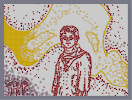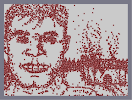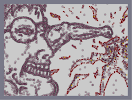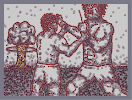HP, A Tribute To Thee Hogwarts Is Never More *2008*John McCain*2008* The Enraged Sailor Harry and the Potter Palemoon vs. The Potter

Pages: (11) [ 1 ] 2 3 4 5 6 7 8 9 10 11

### this

has been my background image for a long time... well done sir
5aved

### Congratulations!!!

This map won the award N-art of the year.

### yeah

numa's startin' to hate N-arts. Except from a few masters like you =D

Congrats on your win. This is still amazing after all this time <(^.^)>

### CONGRATS!!

n-art of the year... and i think ti deserved it. 5/5

.5

### how the hell can u only give 4/5 for this?

its 100% worth 5/5. GJ

5/5.

=D

### I wish I were a reviewer.

I would feature this.

### wow!

well, that´s awesome!

### *salutes*

Next Round!
Will this happen again?
god dammit you made palemoon cry

### *salutes*

*wipes away a single tear*

Everyone

### Haha

NO ONE RATE, its perfect now

Congrats.
officially 2nd page. ouch!
250!

asdf
LOL

### its officially been second-paged

and so close to the finish line ;_;

### two. more. rates.

... well my three days of glory

### oh how it was sidke

it was my moment of glory
"3 days, 14 hours, 41 minutes, and 58 seconds ago."
It was a good run, Espada.

### I'm sick of looking at this

>.<
Oh noez. Sorry espada ;___; but now i assume my map will get sniped because it is at the top

### Once we get to 250

let's let this thing fall people

NOOOOOO!!!

>.<

WOOO!!!!

### Even more impressive.

Is that 300 maps have been submitted after this, yet it's still on top.
BACK UP!!!

### NOOOOOO!!!???!?!?!

IT'S BACK DOWN!!!

### YESS!

GOOD WORK PALEMOON!